• catboost使用
千次阅读
2020-08-11 18:11:18

惊呆了，这么多参数，见鬼。

import catboost as cb


## 参数

loss_function损失函数， 可选RMSE，Logloss，MAE，CrossEntropy
random_seed随机性种子
one_hot_max_size 是否对某些特征进行one-hot编码
custom_metric 自定义监控指标， 可选RMSE，Logloss，MAE，CrossEntropy，Recall，Precision，F1，Accuracy，AUC，R2（具体怎么用我还不会）
eval_metric评价方法， 可选RMSE，Logloss，MAE，CrossEntropy，Recall，Precision，F1，Accuracy，AUC，R2
nan_mode处理NAN的方法，可选Forbidden ，Min，max

iterations tree的数量
learning_rate 学习率
depth树的深度
l2_leaf_reg L2正则化系数机器学习
更多相关内容
• 在JupyterNotebook里使用catboost进行分类训练的时候，当不使用GPU加速时一切正常，而当使用GPU加速时总是会让JupyterNotebook的服务挂掉，这是为什么？ 使用pycharm运行时也正常 ...python 机器学习 数据挖掘
• CatBoost（categorical boosting）是一种能够很好地处理类别型特征的梯度提升算法库。本文中，我们对 CatBoost 基本原理及应用实例做个详细介绍。后面小猴子还将针对其中几个重要特性做专门介绍，如 CatBoost 对类别...

## 梯度提升概述

要理解 boosting，我们首先理解集成学习，为了获得更好的预测性能，集成学习结合多个模型（弱学习器）的预测结果。它的策略就是大力出奇迹，因为弱学习器的有效组合可以生成更准确和更鲁棒的模型。集成学习方法分为三大类：

• Bagging：该技术使用随机数据子集并行构建不同的模型，并聚合所有预测变量的预测结果。

• Boosting：这种技术是可迭代的、顺序进行的和自适应的，因为每个预测器都是针对上一个模型的错误进行修正。

• Stacking：这是一种元学习技术，涉及结合来自多种机器学习算法的预测，例如 bagging 和 boosting。

## 什么是 CatBoost

CatBoost（categorical boosting）是 Yandex 开源的机器学习算法。它可以与深度学习框架轻松集成。它可以处理多种数据类型，以帮助解决企业今天面临的各种问题。CatBoost 和 XGBoost、LightGBM 并称为 GBDT 的三大主流神器，都是在 GBDT 算法框架下的一种改进实现。XGBoost 被广泛的应用于工业界，LightGBM 有效的提升了 GBDT 的计算效率，而 Yandex 的 CatBoost 号称是比 XGBoost 和 LightGBM 在算法准确率等方面表现更为优秀的算法。

CatBoost 是一种基于 对称决策树（oblivious trees）为基学习器实现的参数较少、支持类别型变量和高准确性的GBDT框架，主要解决的痛点是高效合理地处理类别型特征，这一点从它的名字中可以看出来，CatBoost 是由 CategoricalBoosting 组成。此外，CatBoost 还解决了梯度偏差（Gradient Bias）以及预测偏移（Prediction shift）的问题，从而减少过拟合的发生，进而提高算法的准确性和泛化能力。

此外，CatBoost 梯度提升算法库中的学习算法基于 GPU 实现，打分算法基于 CPU 实现。

## CatBoost 的主要特点

CatBoost 优于同类产品的一些关键特性：

### 01 对称树

与 XGBoost 和 LightGBM 不同，CatBoost 构建对称（平衡）树。在每一步中，前一棵树的叶子都使用相同的条件进行拆分。选择损失最低的特征分割对并将其用于所有级别的节点。这种平衡的树结构有助于高效的 CPU 实现，减少预测时间，模型结构可作为正则化以防止过度拟合。

在对称决策树中，只使用一个特性来构建每个树级别上的所有分支。我们使用图示方法更加清晰地观察三种类型的拆分："FloatFeature"、"OneHotFeature" 和 "OnlineCtr"

#### FloatFeature

模型没有类别型特征时，在可视化的树中只有 "FloatFeature" 节点。"FloatFeature" 拆分对应的节点包含特征索引和边界值，用于拆分对象。

boston = load_boston()
y = boston['target']
X = boston['data']
pool = catboost.Pool(data=X, label=y)
model = CatBoostRegressor(depth=2, verbose=False, iterations=1).fit(X, y)
model.plot_tree(tree_idx=0,
# pool=pool, )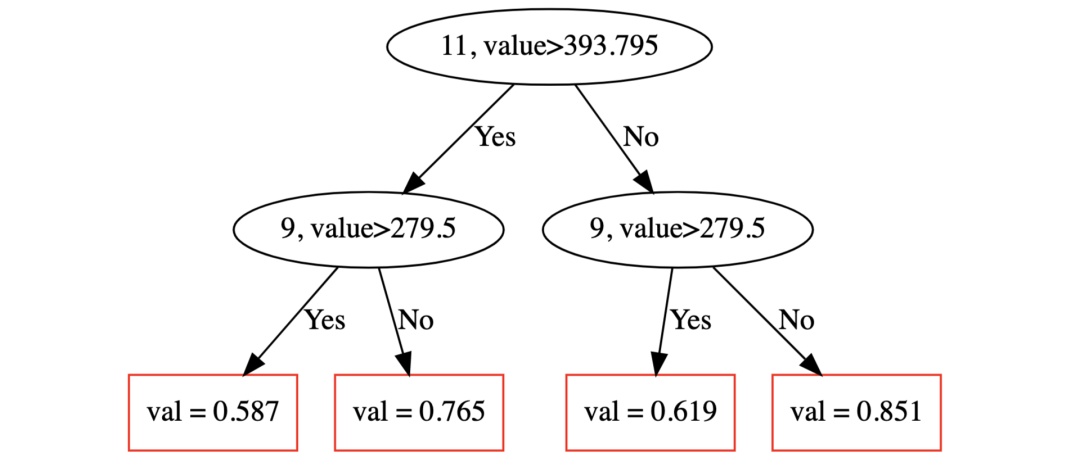在这个例子中，深度为0的节点表示对象被它们的第0个带边界值的特征分割。类似地，深度1的节点通过其具有边界值的第二个特征来分割对象。

#### OneHotFeature

titanic_df = titanic()

X = titanic_df.drop('Survived',axis=1)
y = titanic_df.Survived
# 分类变量的缺失值用"NAN"填充，代码略
pool = Pool(X, y, cat_features=cat_features_index, feature_names=list(X.columns))
model = CatBoostClassifier(
max_depth=2, verbose=False, max_ctr_complexity=1, random_seed=42, iterations=2).fit(pool)
model.plot_tree(
tree_idx=0,
pool=pool # 对于一个需要使用独热编码的特征，"pool" 是一个必须的参数
)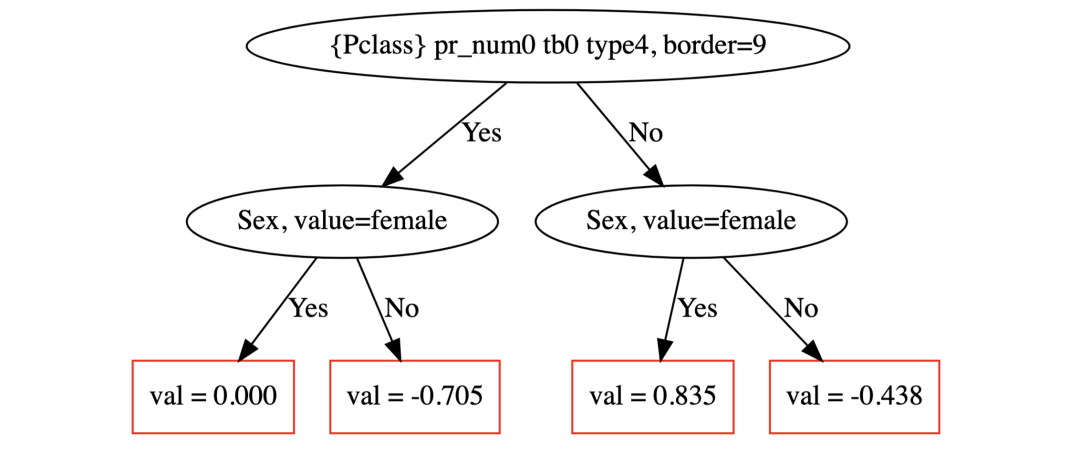第一棵树只包含一个由"OneHotFeature"特征产生的分裂。这种分割将"Sex=female"的对象放在左边，而"other"的对象放在右边。

#### OnlineCtr

model.plot_tree(tree_idx=1, pool=pool)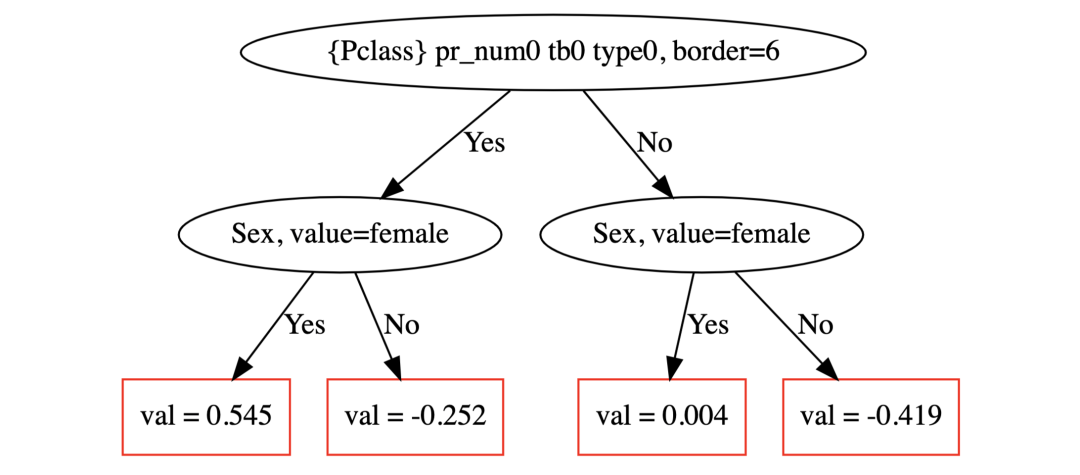### 02 Ordered Boosting

经典提升算法存在预测偏移的问题，容易在小的/嘈杂的数据集上过度拟合。在计算数据实例的梯度估计时，这些算法使用与构建模型相同的数据实例，因此没有机会遇到看不见的数据。

另一方面，CatBoost 使用排序提升的概念，这是一种置换驱动的方法，在数据子集上训练模型，同时在另一个子集上计算残差，从而防止目标泄漏和过度拟合。

### 03 鲁棒性

它减少了对大量超参数调整的需求，并降低了过度拟合的机会，这也导致了更通用的模型。虽然，CatBoost 有多个参数需要调整，它包含树的数量、学习率、正则化、树深度、折叠大小、装袋温度等参数。您可以在此处阅读所有这些参数。

### 04 原生特征支持,易于使用

CatBoost 支持数字、分类或文本的各种特征，节省了预处理的时间和精力。可以从命令行使用 CatBoost，使用 Python 和 R 的用户友好 API。

## CatBoost 的基本使用

### 导入基本数据

我们使用 CatBoost 库里自带的经典数据集 titanic。

from catboost.datasets import titanic
import numpy as np
train_df, test_df = titanic()
# 用一些超出分布范围的数字来填充缺失值
train_df.fillna(-999, inplace=True)
test_df.fillna(-999, inplace=True)
# 拆分特征变量及标签变量
X = train_df.drop('Survived', axis=1)
y = train_df.Survived
# 划分训练集和测试集
from sklearn.model_selection import train_test_split
X_train, X_validation, y_train, y_validation = train_test_split(X, y, train_size=0.75, random_state=42)
X_test = test_df

Titanic 数据集中的特征变量具有不同的类型—有些是数值类型，有些是分类类型，甚至有些只是字符串类型，通常应该以某种特定的方式进行处理(例如使用词袋表示进行编码)。但这里我们可以将这些字符串特征视为分类特征——所有繁重的工作都是在 CatBoost 中完成的。

### 创建一个baseline模型

我们首先从一个基础baseline模型开始，认识如何使用catboost进行预测目标。

# !pip install catboost
from catboost import CatBoostClassifier, Pool, metrics, cv
from sklearn.metrics import accuracy_score

model = CatBoostClassifier(
custom_loss=[metrics.Accuracy()], # 该指标可以计算logloss，并且在该规模的数据集上更加光滑
random_seed=42,
logging_level='Silent'
)
# 模型训练
model.fit(
X_train, y_train,
cat_features=categorical_features_indices,
eval_set=(X_validation, y_validation),
#     logging_level='Verbose',  # you can uncomment this for text output
plot=True
);

可以通过详细输出或使用漂亮的绘图来观察我们的模型学习，我们使用CatBoost自带的可交互模型过程可视化，查看模型的学习过程。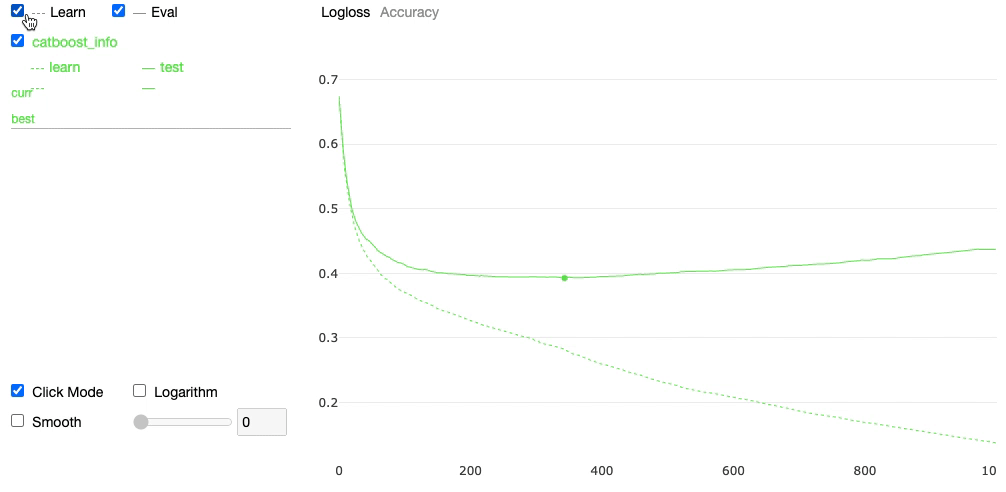### 特征变量统计

#### Float feature

feature = 'Fare'
res = model.calc_feature_statistics(
X_train, y_train, feature, plot=True)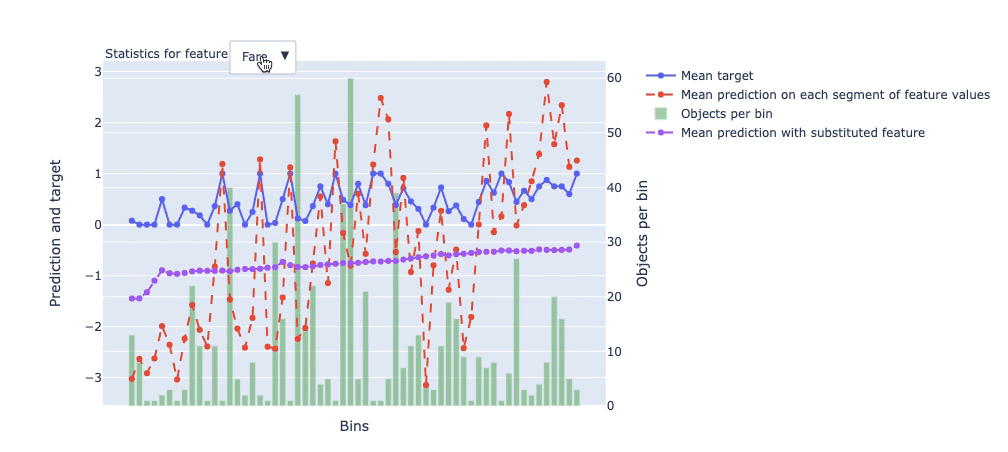#### One-hot feature

feature = 'Sex'
res = model.calc_feature_statistics(X_train, y_train, feature, plot=True)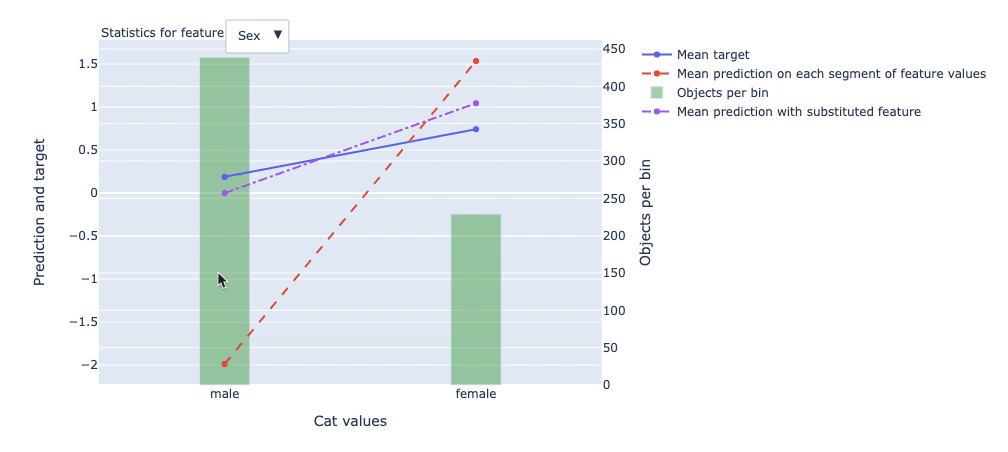### 模型交叉验证

验证模型是否是性能最佳的，使用交叉验证，可能甚至会得到更好的模型。

cv_params = model.get_params()
cv_params.update({
'loss_function': metrics.Logloss()
})
cv_data = cv(
Pool(X, y, cat_features=categorical_features_indices),
cv_params,
plot=True
)现在我们得到了每个 boosting 步骤 3folds 平均的损失函数值，这提供了更准确的模型性能估计。

print('Best validation accuracy score: {:.2f}±{:.2f} on step {}'.format(
np.max(cv_data['test-Accuracy-mean']),
cv_data['test-Accuracy-std'][np.argmax(cv_data['test-Accuracy-mean'])],
np.argmax(cv_data['test-Accuracy-mean'])
))

print('Precise validation accuracy score: {}'.format(np.max(cv_data['test-Accuracy-mean'])))
Best validation accuracy score:
0.83±0.02 on step 355
Precise validation accuracy score:
0.8294051627384961

如上所示，最初对单次验证的性能估计并不是特别理想，而经过交叉验证后会有所提升。

### 模型应用

模型训练结束后，可以保存模型，以供预测使用。

predictions = model.predict(X_test)
predictions_probs = model.predict_proba(X_test)
print(predictions[:10])
print(predictions_probs[:10])
[0 0 0 0 1 0 1 0 1 0]
[[0.85473931 0.14526069]
[0.76313031 0.23686969]
[0.88972889 0.11027111]
[0.87876173 0.12123827]
[0.3611047  0.6388953 ]
[0.90513381 0.09486619]
[0.33434185 0.66565815]
[0.78468564 0.21531436]
[0.39429048 0.60570952]
[0.94047549 0.05952451]]

## CatBoost 应用案例

出于演示目的，我们同样使用 catboost 自带的 amazon 数据集。

import pandas as pd
import os
import numpy as np
np.set_printoptions(precision=4)
import catboost
from catboost import *
from catboost import datasets

(train_df, test_df) = catboost.datasets.amazon()
train_df.head()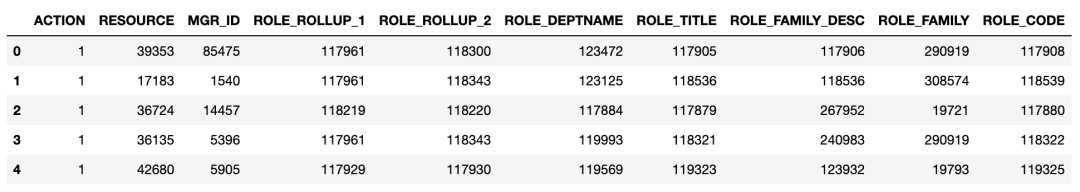### 数据预处理

##### 数据标签提取
y = train_df.ACTION
X = train_df.drop('ACTION', axis=1)
##### 检查数据集中标签平衡性
print('Labels: {}'.format(set(y)))
print('Zero count = {}, One count = {}'.format(len(y) - sum(y), sum(y)))
abels: {0, 1}
Zero count = 1897, One count = 30872
##### 保存数据
dataset_dir = './amazon'
if not os.path.exists(dataset_dir):
os.makedirs(dataset_dir)

train_df.to_csv(
os.path.join(dataset_dir, 'train.csv'),
)
test_df.to_csv(
os.path.join(dataset_dir, 'test.csv'),
)
##### 创建 Pool 类
from catboost.utils import create_cd
feature_names = dict()
for column, name in enumerate(train_df):
if column == 0:
continue
feature_names[column - 1] = name

create_cd(
label=0,
cat_features=list(range(1, train_df.columns.shape)),
feature_names=feature_names,
output_path=os.path.join(dataset_dir, 'train.cd')
)

!cat amazon/train.cd
0	Label
1	Categ	RESOURCE
2	Categ	MGR_ID
3	Categ	ROLE_ROLLUP_1
4	Categ	ROLE_ROLLUP_2
5	Categ	ROLE_DEPTNAME
6	Categ	ROLE_TITLE
7	Categ	ROLE_FAMILY_DESC
8	Categ	ROLE_FAMILY
9	Categ	ROLE_CODE

这里展示了几种创建Pool的不同方法，实际中你选中其中一种创建方法即可。

pool1 = Pool(data=X, label=y, cat_features=cat_features)
pool2 = Pool(
data=os.path.join(dataset_dir, 'train.csv'),
delimiter=',',
column_description=os.path.join(dataset_dir, 'train.cd'),
)
pool3 = Pool(data=X, cat_features=cat_features)

# 创建Pool的最快方法是从numpy矩阵创建它。
# 如果你想要快速的预测或者以最快的方式在python中加载数据，就应该使用这种方式。

X_prepared = X.values.astype(str).astype(object)
# 对于FeaturesData类，类别特性必须具有str类型

pool4 = Pool(
data=FeaturesData(
cat_feature_data=X_prepared,
cat_feature_names=list(X)
),
label=y.values
)

print('Dataset shape')
print('dataset 1:' + str(pool1.shape) +
'\ndataset 2:' + str(pool2.shape) +
'\ndataset 3:' + str(pool3.shape) +
'\ndataset 4:' + str(pool4.shape))

print('\n')
print('Column names')
print('dataset 1:')
print(pool1.get_feature_names())
print('\ndataset 2:')
print(pool2.get_feature_names())
print('\ndataset 3:')
print(pool3.get_feature_names())
print('\ndataset 4:')
print(pool4.get_feature_names())
Dataset shape
dataset 1:(32769, 9)
dataset 2:(32769, 9)
dataset 3:(32769, 9)
dataset 4:(32769, 9)

Column names
dataset 1:
['RESOURCE', 'MGR_ID', 'ROLE_ROLLUP_1',
'ROLE_ROLLUP_2', 'ROLE_DEPTNAME',
'ROLE_TITLE', 'ROLE_FAMILY_DESC',
'ROLE_FAMILY', 'ROLE_CODE']

dataset 2:
['RESOURCE', 'MGR_ID', 'ROLE_ROLLUP_1',
'ROLE_ROLLUP_2', 'ROLE_DEPTNAME',
'ROLE_TITLE', 'ROLE_FAMILY_DESC',
'ROLE_FAMILY', 'ROLE_CODE']

dataset 3:
['RESOURCE', 'MGR_ID', 'ROLE_ROLLUP_1',
'ROLE_ROLLUP_2', 'ROLE_DEPTNAME',
'ROLE_TITLE', 'ROLE_FAMILY_DESC',
'ROLE_FAMILY', 'ROLE_CODE']

dataset 4:
['RESOURCE', 'MGR_ID', 'ROLE_ROLLUP_1',
'ROLE_ROLLUP_2', 'ROLE_DEPTNAME',
'ROLE_TITLE', 'ROLE_FAMILY_DESC',
'ROLE_FAMILY', 'ROLE_CODE']

### 拆分训练集和测试集

这一步大家应该比较熟悉，就不做过多的介绍。

from sklearn.model_selection import train_test_split
X_train, X_validation, y_train, y_validation = train_test_split(
X, y, train_size=0.8, random_state=1234)

### 选择目标函数

对于二分类数据集，目标函数可以选择Logloss，如果要预测目标标签的概率值，推荐使用交叉熵CrossEntropy

from catboost import CatBoostClassifier
model = CatBoostClassifier(
iterations=5,
learning_rate=0.1,
# loss_function='CrossEntropy'
)
model.fit(
X_train, y_train,
cat_features=cat_features,
eval_set=(X_validation, y_validation),
verbose=False
)
print('Model is fitted: ' + str(model.is_fitted()))
print('Model params:')
print(model.get_params())
Model is fitted: True
Model params:
{'iterations': 5, 'learning_rate': 0.1}

### 训练模型

模型训练与通常sklearn模型训练差异不大，先实例化模型 model，然后直接 fit 训练即可。

from catboost import CatBoostClassifier
model = CatBoostClassifier(
iterations=15,
#     verbose=5,
)
model.fit(
X_train, y_train,
cat_features=cat_features,
eval_set=(X_validation, y_validation),
)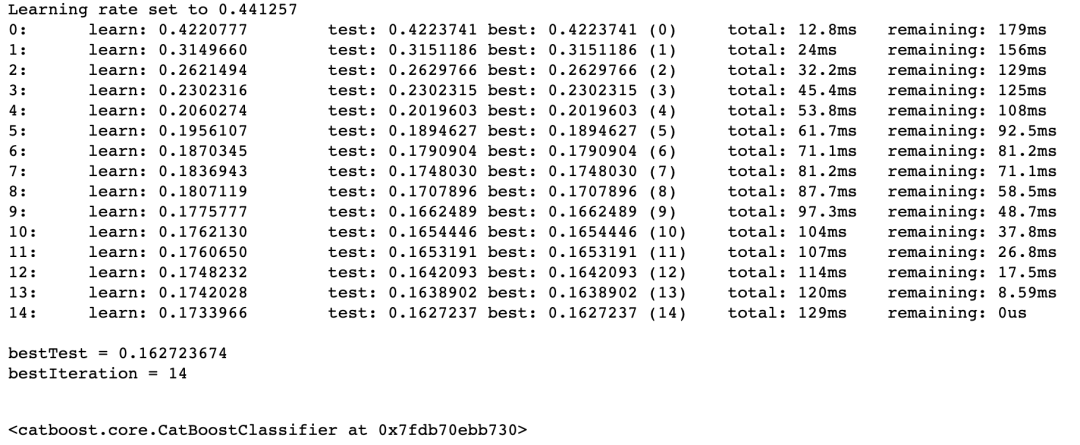### 评估模型

Catboost 做模型评估时，同一般模型少有区别，该模型在 model.fit() 时，传递给参数 eval_set 相应的验证子集，设置参数 plotTrue，即可在训练模型的同时，用验证集评估模型，并且输出过程可视化结果，可谓是非常方便与惊艳。

from catboost import CatBoostClassifier
model = CatBoostClassifier(
iterations=50,
random_seed=63,
learning_rate=0.5,
custom_loss=['AUC', 'Accuracy']
)
model.fit(
X_train, y_train,
cat_features=cat_features,
eval_set=(X_validation, y_validation),
verbose=False,
plot=True
)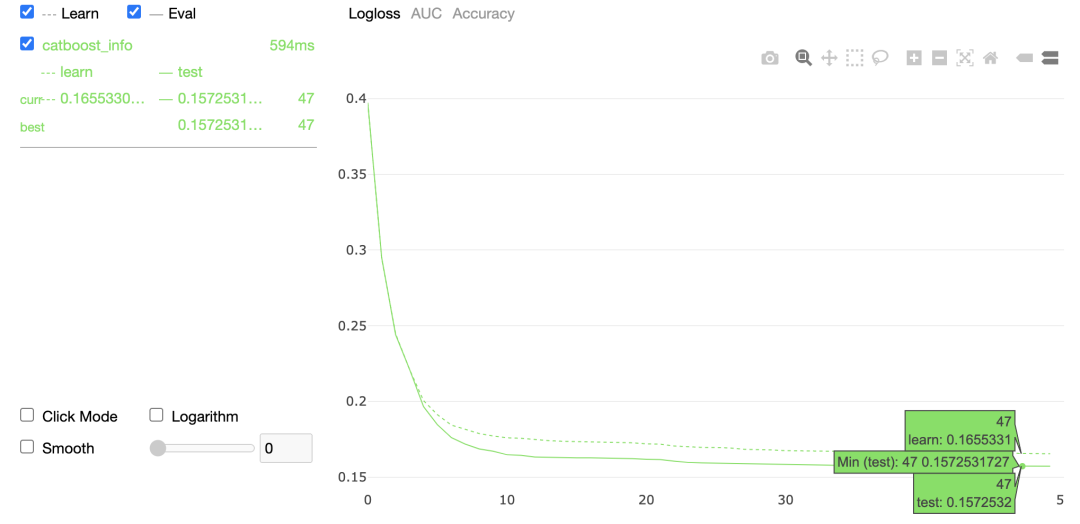### 模型比较

与模型评估一样，使用相同 CatBoostClassifier 分类器，仅仅设置不同的 learning_rate，并设置train_dir分别为 'learing_rate_0.7''learing_rate_0.01'

model1 = CatBoostClassifier(
learning_rate=0.7,
iterations=100,
random_seed=0,
train_dir='learing_rate_0.7'
)

model2 = CatBoostClassifier(
learning_rate=0.01,
iterations=100,
random_seed=0,
train_dir='learing_rate_0.01'
)
model1.fit(
X_train, y_train,
eval_set=(X_validation, y_validation),
cat_features=cat_features,
verbose=False
)
model2.fit(
X_train, y_train,
eval_set=(X_validation, y_validation),
cat_features=cat_features,
verbose=False
)

然后使用catboost的MetricVisualizer方法比较两个模型。该方法在单个图表上绘制有关训练、指标评估或交叉验证运行的信息。根据输入信息，一个图表可以包含有关一次或多次运行的信息。图表既可以在训练进行时实时绘制，也可以在训练结束后绘制。

from catboost import MetricVisualizer
MetricVisualizer(['learing_rate_0.01', 'learing_rate_0.7']).start()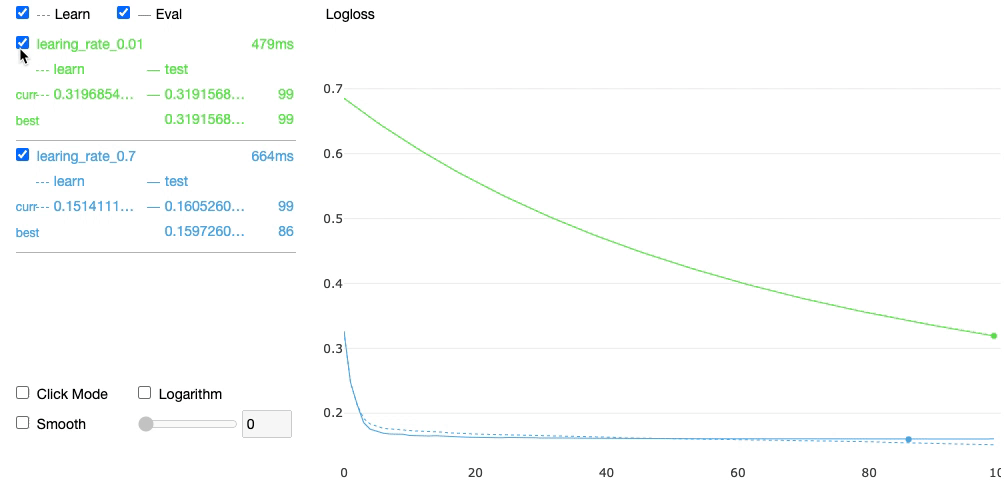### 交叉验证

在前面已经提到，使用交叉验证可以得到性能更好的模型，进而得到更好的预测结果。相对使用sklearn 中的交叉验证方法，Catboost 模型自带的交叉验证方法简单、灵活，还可以直接显示可视化交叉验证过程及结果。下面小猴子录制了动画，展示交叉验证过程。

from catboost import cv
# 设置参数空间
params = {}
params['loss_function'] = 'Logloss'
params['iterations'] = 80
params['custom_loss'] = 'AUC'
params['random_seed'] = 63
params['learning_rate'] = 0.5
# 直接使用catboost中自带的cv参数。
cv_data = cv(
params = params,
pool = Pool(X, label=y, cat_features=cat_features), # 设置Pool类。
fold_count=5,
shuffle=True,
partition_random_seed=0,
plot=True,   # 设置可视化过程
stratified=False,
verbose=False
)交叉验证过程中所有数据都记录下来并以DataFrame格式返回，可以直接查看，或后续使用，非常方便！

cv_data.head()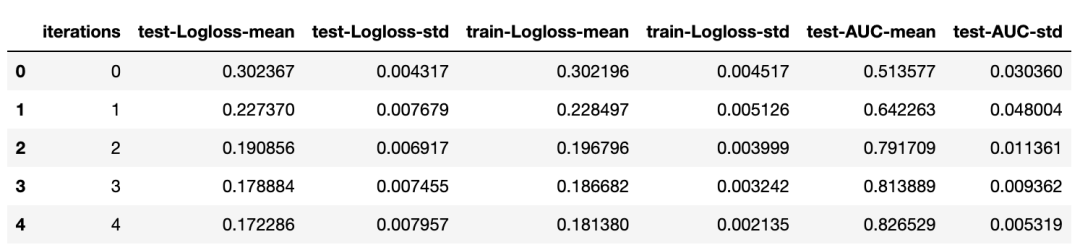其实，我们只关系最佳得分，使用如下方法可以轻松获得：

best_value = np.min(cv_data['test-Logloss-mean'])
best_iter = np.argmin(cv_data['test-Logloss-mean'])

print('Best validation Logloss score, not stratified: {:.4f}±{:.4f} on step {}'.format(
best_value,
cv_data['test-Logloss-std'][best_iter],
best_iter)
)
Best validation Logloss score,
not stratified: 0.1581±0.0104 on step 52

### 过拟合检验

在创建CatBoostClassifier实例时，设置参数early_stopping_rounds=20（根据实际情况设置），模型可以在 early_stopping_rounds 所设置的迭代轮数内寻找模型效果最好的，这个模型效果评价指标可以通过eval_metric设置，默认 Logloss，也可以设置为"AUC"。还可以通过设置custom_metric参数，使用自定义评价指标函数。

model_with_early_stop = CatBoostClassifier(
eval_metric='AUC',
iterations=200,
random_seed=63,
learning_rate=0.5,
early_stopping_rounds=20
)
model_with_early_stop.fit(
X_train, y_train,
cat_features=cat_features,
eval_set=(X_validation, y_validation),
verbose=False,
plot=True
)print(model_with_early_stop.tree_count_)
30

可以使用tree_count_属性查看在何时停止的。

### 选择概率决策边界

#### 绘制 ROC 曲线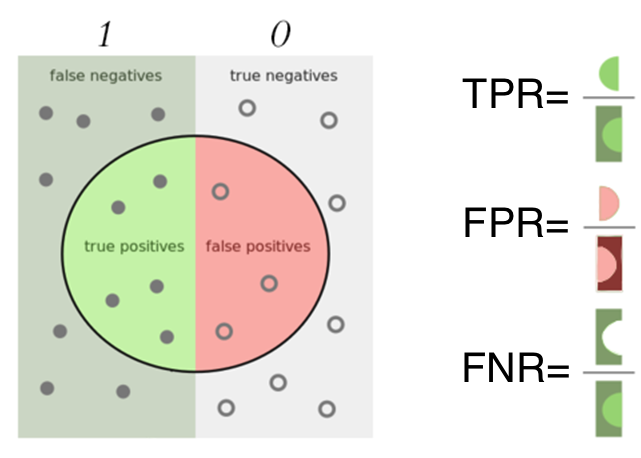首先使用catboost的工具函数get_roc_curve获取到在验证池中的数据fpr 和  tpr 值，然后将其输入到 sklearn 中的 auc 函数中，计算得到 roc_auc 面积大小。为了更加直观，我们绘制如下曲线。

from catboost.utils import get_roc_curve
import sklearn
from sklearn import metrics

eval_pool = Pool(X_validation, y_validation, cat_features=cat_features)
curve = get_roc_curve(model, eval_pool)
(fpr, tpr, thresholds) = curve
roc_auc = sklearn.metrics.auc(fpr, tpr)

lw = 2
plt.plot(fpr, tpr, color='darkorange',
lw=lw, label='ROC curve (area = %0.2f)' % roc_auc, alpha=0.5)
plt.plot([0, 1], [0, 1], color='navy', lw=lw, linestyle='--', alpha=0.5)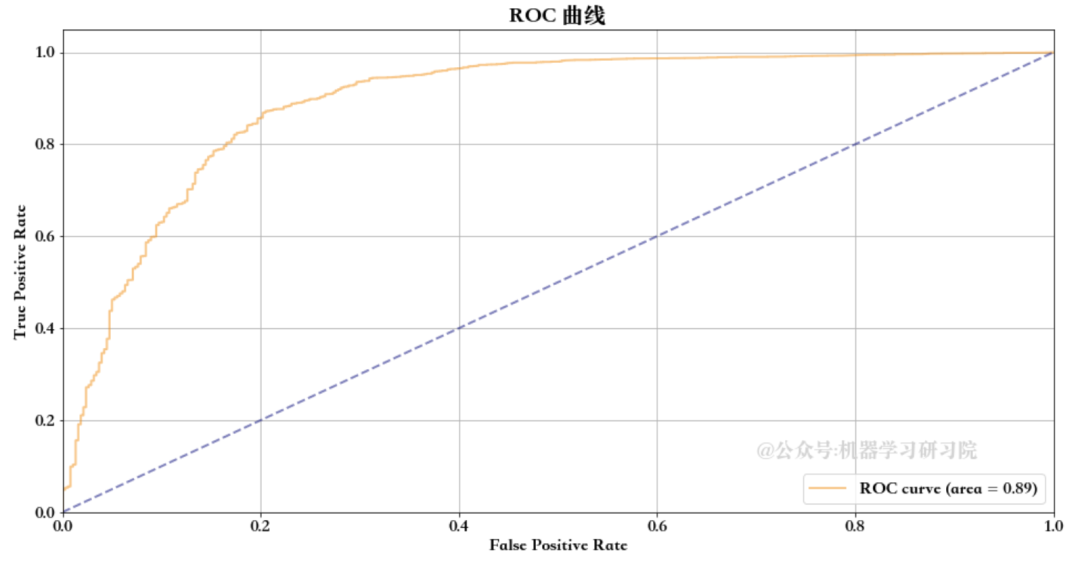除了上面上面用于ROC曲线的FPR，TPR，另外还可以绘制FPR，FNR曲线。

from catboost.utils import get_fpr_curve
from catboost.utils import get_fnr_curve

(thresholds, fpr) = get_fpr_curve(curve=curve)
(thresholds, fnr) = get_fnr_curve(curve=curve)
lw = 2
plt.plot(thresholds, fpr, color='blue', lw=lw, label='FPR', alpha=0.5)
plt.plot(thresholds, fnr, color='green', lw=lw, label='FNR', alpha=0.5)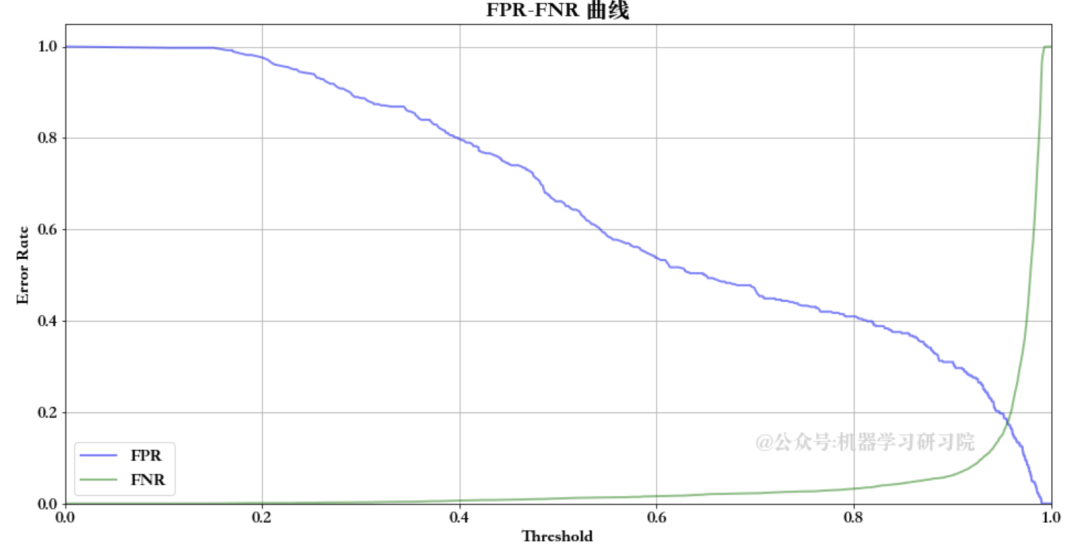返回实现指定FNR或FPR所需的概率边界。

from catboost.utils import select_threshold

print(select_threshold(model=model, data=eval_pool, FNR=0.01))
print(select_threshold(model=model, data=eval_pool, FPR=0.01))
0.48689529945049076
0.9899713850692811

### 模型预测

CatBoost预测有四种方式，predict、staged_predict、predict_proba 及 staged_predict_prob。我们看下他们之间的区别。

首先 predictpredict_proba，将模型应用于给定数据集，预测得到结果，predict 是直接得到计算后的结果，如果是二分类，就是0或1。predict_proba 结果是归属于哪种类别的概率值。

print(model.predict_proba(X=X_validation))
[[0.0608 0.9392]
[0.0141 0.9859]
[0.0126 0.9874]
...
[0.0148 0.9852]
[0.0215 0.9785]
[0.0333 0.9667]]
print(model.predict(data=X_validation))
[1 1 1 ... 1 1 1]

与常规预测不同，Predict()  函数中有个 prediction_type 参数，支持的预测类型包含多种：

• Probability

• Class

• RawFormulaVal

• Exponent

• LogProbability

raw_pred = model.predict(
data=X_validation,
prediction_type='RawFormulaVal'
)
print(raw_pred)
[2.7374 4.2445 4.3614 ... 4.1992 3.8198 3.3681]

可以通过 Sigmoid 函数将上面结果转换为概率。

from numpy import exp
sigmoid = lambda x: 1 / (1 + exp(-x))
probabilities = sigmoid(raw_pred)
print(probabilities)
[0.9392 0.9859 0.9874 ... 0.9852 0.9785 0.9667]

另一个就是 staged_predict 及 staged_predict_prob，他是阶段预测，仅考虑 trees 在range[0; i) 内的计算结果值。这个范围是通过参数

eval_period控制的：

要在应用模型或计算指标时减少要使用的树的数量，将树索引的范围设置为[ntree_start; ntree_end)并将要使用的树的步长设置为eval_period

此参数定义迭代范围的步骤[ntree_start; ntree_end)。例如，假设设置了以下参数值：

• ntree_start设置为 0

• ntree_end设置为 N（总树数）

• eval_period设置为 2

在这种情况下，将返回以下树范围的结果：[0, 2), [0, 4), ... , [0, N)

predictions_gen = model.staged_predict_proba(
data=X_validation,
ntree_start=0,
ntree_end=5,
eval_period=1
)
try:
for iteration, predictions in enumerate(predictions_gen):
print('Iteration ' + str(iteration) + ', predictions:')
print(predictions)
except Exception:
pass
Iteration 0, predictions:
[[0.3726 0.6274]
...
[0.3726 0.6274]]
...
Iteration 4, predictions:
[[0.1388 0.8612]
...
[0.175  0.825 ]]

### 在未知数据集上评估模型

我们使用 eval_metrics 方法计算指定数据集的指定指标。

metrics = model.eval_metrics(
data=pool1,
metrics=['Logloss','AUC'],
ntree_start=0,
ntree_end=0,
eval_period=1,
plot=True
)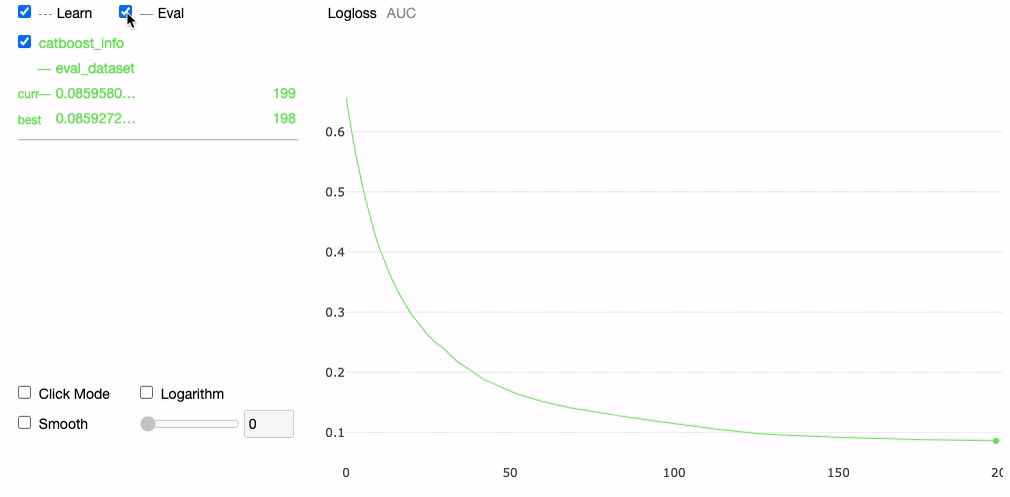从可视化结果看，eval_metrics 只包含 Eval 结果曲线，我们设置了  metrics=['Logloss','AUC'] ，因此包含'Logloss''AUC'两条评估曲线。

print('AUC values:')
print(np.array(metrics['AUC']))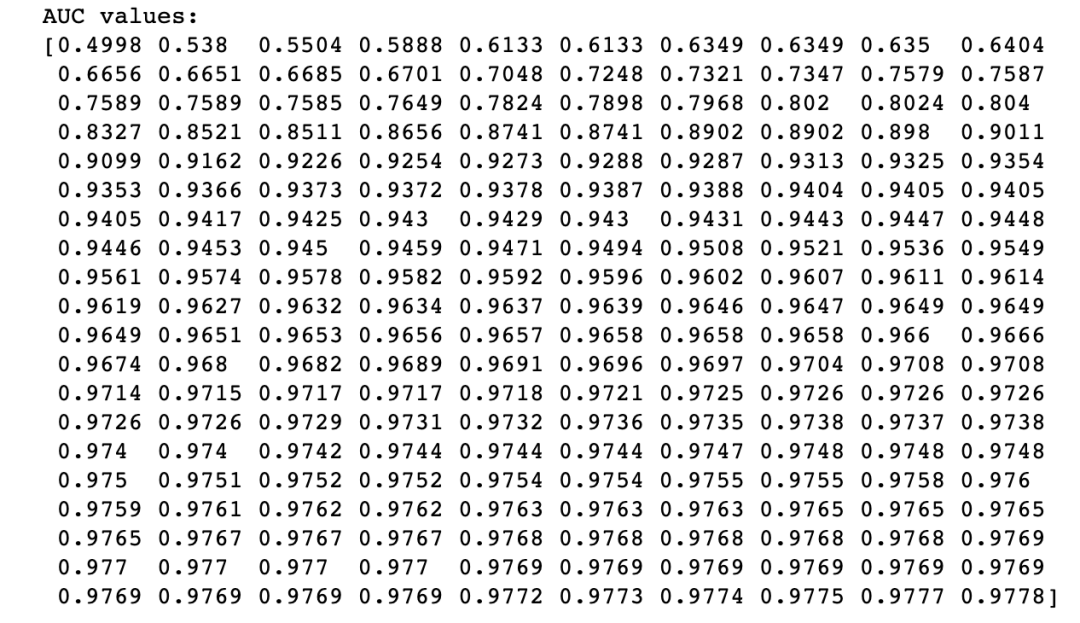### 特征重要性

使用模型自带的get_feature_importance方法。

model.get_feature_importance(prettified=True)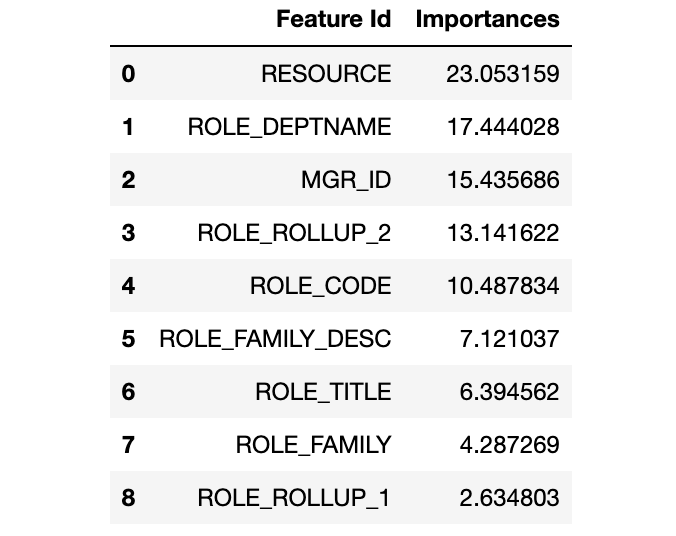使用第三方解释库 Shap。与一般模型直接使用 Shap 有所不同，使用 model.get_feature_importance() 方法，并设置参数 type='ShapValues'， 直接输出 shap_values 值，该值可直接用户输出结果值及绘制相应可视化图形。

shap_values = model.get_feature_importance(
pool1, type='ShapValues')
expected_value = shap_values[0,-1]
shap_values = shap_values[:,:-1]
print(shap_values.shape)

(32769, 9)

import shap

shap.initjs()
shap.force_plot(expected_value, shap_values[3,:], X.iloc[3,:])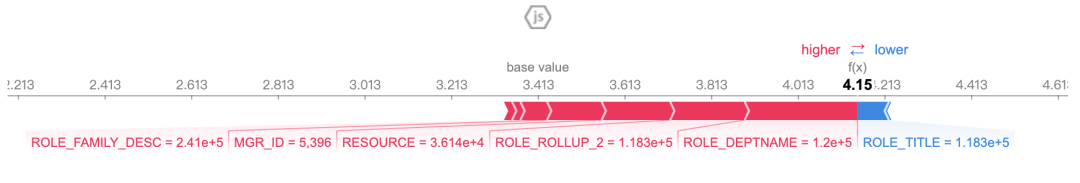shap.initjs()
shap.force_plot(expected_value, shap_values[91,:], X.iloc[91,:])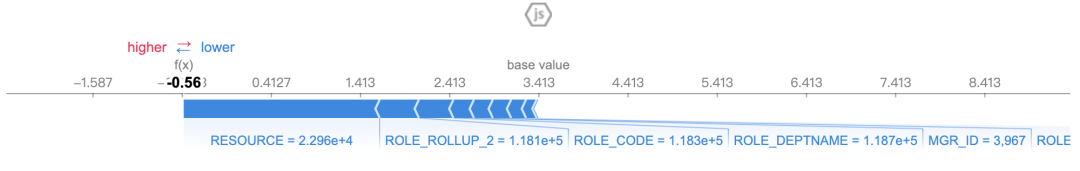shap.summary_plot(shap_values, X)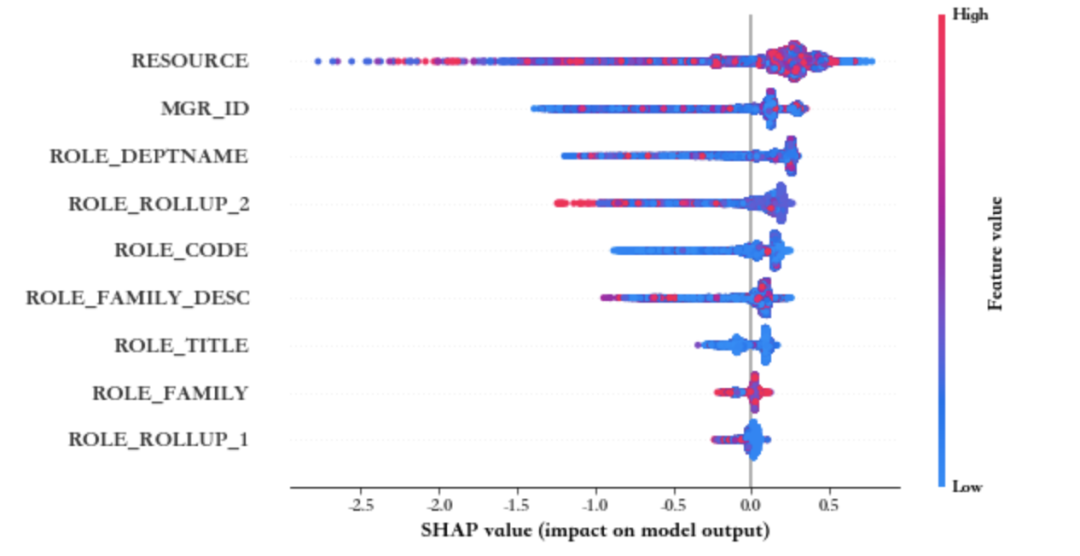X_small = X.iloc[0:200]
shap_small = shap_values[:200]
shap.force_plot(expected_value, shap_small, X_small)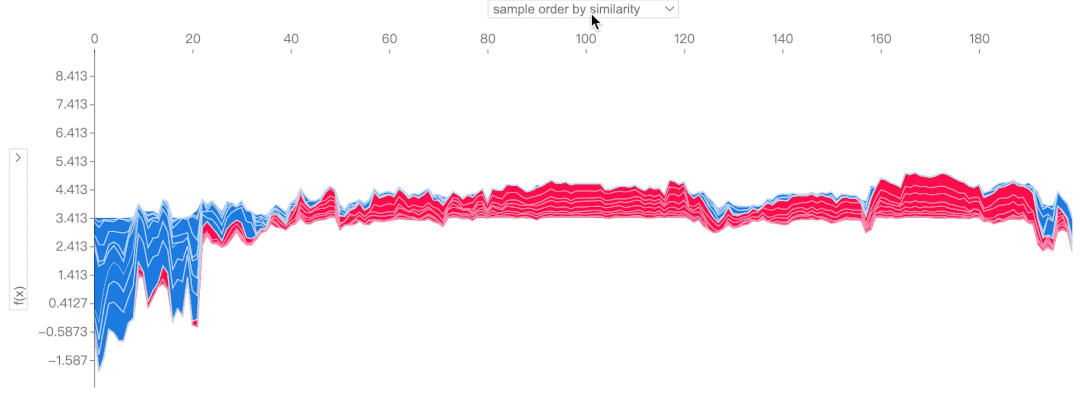### 特征评估

CatBoost还有个很厉害的功能，就是对指定特征进行评估，给出评估结果，是好是坏

from catboost.eval.catboost_evaluation import *
learn_params = {'iterations': 20, # 2000
'learning_rate': 0.5, # we set big learning_rate,
# because we have small
# #iterations
'random_seed': 0,
'verbose': False,
'loss_function' : 'Logloss',
'boosting_type': 'Plain'}
evaluator = CatboostEvaluation(
'amazon/train.tsv',
fold_size=10000, # <= 50% of dataset
fold_count=20,
column_description='amazon/train.cd',
partition_random_seed=0,
#working_dir=...
)
result = evaluator.eval_features(
learn_config=learn_params,
eval_metrics=['Logloss', 'Accuracy'],
features_to_eval=[6, 7, 8])

以上设定用来评估的特征是[6, 7, 8]，从以下结果看到特征6得到正向结论，而特征8得到负向结论，特征7从各项指标中得不到确切的指标。

from catboost.eval.evaluation_result import *
logloss_result = result.get_metric_results('Logloss')
logloss_result.get_baseline_comparison(
ScoreConfig(ScoreType.Rel, overfit_iterations_info=False)
)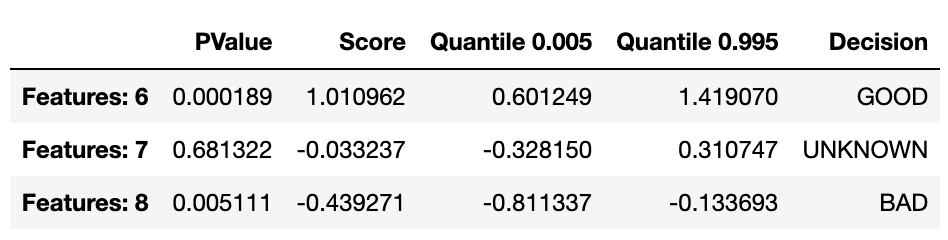### 模型保存和导入

当我们得到一个较为理想的模型后，需要保存模型，以后期使用模型，因此，该步骤还是非常重要的。而CatBoost保存模型非常方便，无需借助第三方库如pickle等，直接使用其save_model方法，即可保存模型。

save_model保存模型，可以保存为各种格式：

• cbm — CatBoost 二进制格式。

• coreml — Apple CoreML 格式（目前仅支持没有分类特征的数据集）。

• json — JSON 格式。有关格式详细信息，请参阅CatBoost JSON 模型教程

• python — 独立的 Python 代码（目前不支持多分类模型）。有关应用结果模型的详细信息，请参阅 Python部分。

• cpp — 独立 C++ 代码（当前不支持多分类模型）。有关应用结果模型的详细信息，请参阅 C++部分。

• onnx — ONNX-ML 格式（目前仅支持没有分类特征的数据集）。详情请参阅 https://onnx.ai/。有关应用结果模型的详细信息，请参阅 ONNX部分。

• pmml — PMML 4.3 版格式。如果训练数据集中存在分类特征，则必须在训练期间将其解释为 one-hot 编码。这可以通过将--one-hot-max-size/one_hot_max_size参数设置为大于数据集中所有分类特征中唯一分类特征值的最大数量的值来实现。有关应用结果模型的详细信息，请参阅PMML部分。

my_best_model.save_model('catboost_model.bin')
my_best_model.save_model('catboost_model.json', format='json')

当然，导入模型也是非常方便，直接使用load_model 方法

my_best_model.load_model('catboost_model.bin')
print(my_best_model.get_params())
print(my_best_model.random_seed_)

### 参考资料



CatBoost JSON 模型教程: https://github.com/catboost/tutorials/blob/master/model_analysis/model_export_as_json_tutorial.ipynb



Python: https://catboost.ai/en/docs/concepts/python-reference_apply_catboost_model



C++: https://catboost.ai/en/docs/concepts/c-plus-plus-api_applycatboostmodel



ONNX: https://catboost.ai/en/docs/concepts/apply-onnx-ml



PMML 4.3 版: http://dmg.org/pmml/pmml-v4-3.html



PMML: https://catboost.ai/en/docs/concepts/apply-pmml

推荐阅读：

互联网校招研发薪资汇总

公众号：AI蜗牛车

保持谦逊、保持自律、保持进步发送【蜗牛】获取一份《手把手AI项目》（AI蜗牛车著）

发送【1222】获取一份不错的leetcode刷题笔记

发送【AI四大名著】获取四本经典AI电子书

展开全文大数据 数据挖掘 python
• Kaggle比赛各种增强算法，CatBoost 和 Light GBM 和 XGBoost 每种算法处理类别变量 了解参数 在数据集上实现 每种算法的性能

# 学习目标：

对比学习：CatBoost 和 Light GBM 和 XGBoost

• Light GBM 最快，目前暂时对GPU CUDA支持不好，报错
• CatBoost 有较多离散特征时效果好，对GPU支持好，所以用GPU训练快
• XGBoost最慢，需要自己编码，效果较稳定

# 学习内容：

Kaggle比赛使用各种增强算法，能进入前10名。尽管神经网络最近重新出现并流行起来，但传统提升算法，在训练数据有限，训练时间少，参数调优专业知识少的情况下，仍然更有用。

CatBoost 和 Light GBM 和 XGBoost发展时间表。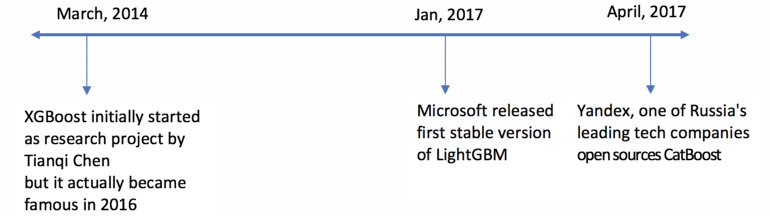由于XGBoost（通常称为GBM Killer）已经在机器学习领域工作了更长的时间，并且有很多文章专门介绍它，因此本文将更多地关注CatBoost和LGBM。以下是我们将涵盖的主题：

• 结构差异
• 每种算法处理类别变量的方式
• 了解调优参数
• 在数据集上具体实现
• 每种算法的性能对比

# LightGBM和XGBoost的结构差异

LightGBM使用基于梯度的单侧采样（GOSS）技术来过滤掉用于查找拆分值的数据，而XGBoost使用预排序算法和基于直方图的算法来计算最佳拆分。

首先，让我们了解一下XGBoost预排序拆分的工作原理：

1、对于每个特征，枚举所有取值
2、对于每个特征，按值对数据进行排序
3、使用线性扫描确定以该特征的信息增益的最佳分割
4、在所有特征上采取最佳的分割方案
lightGBM基于直方图拆分的工作原理：
简单来说，基于直方图的算法将特征的所有取值进行分箱，数据被拆分为离散柱，并使用这些条柱来查找直方图的分割值。因此大大减小了最佳分割点的可能取值，也就是说不用把特征的每个取值都来计算信息增益，只用在离散后的分割点处计算信息增益即可，这也是分箱的精髓。
每个虽然在训练速度上，基于直方图拆分比预排序算法更有效，它枚举了预先排序的特征值上所有可能的分割点，但在速度方面仍然落后于GOSS。

那么，是什么让这种GOSS方法变得高效呢？

梯度表示损失函数正切的斜率，因此从逻辑上讲，如果数据点的梯度在某种意义上很大，则这些点对于找到最佳分割点非常重要，因为它们具有更高的误差。更能帮助损失函数到达最优点。

GOSS 保留所有梯度较大的实例数据，并对梯度较小的实例执行随机抽样。例如，假设我有 500K 行数据，其中 10k 行具有较高的梯度。所以我的算法会选择（10k行的较高梯度+ 剩余490k行的x%随机选择）。假设 x 为 10%，则选择的总行数为 500K 中的 59k，如果找到拆分值，则基于该值。注意，这里随机选取的10%的数据的权重会人为增大9倍，这样才能保证原始数据中，数据的分布一致，不然这样采样会改变原始的数据分布。这点需要理解。

这里采取的基本假设是，具有小梯度的训练实例的样本具有较小的训练误差，并且已经训练有素。
为了保持相同的数据分布，在计算信息增益时，GOSS为梯度较小的数据实例引入了常数乘数。因此，GOSS在减少数据实例数量和保持学习决策树的准确性之间实现了良好的平衡。

具有较高梯度/误差的叶子用于在LGBM中进一步生长，LGBM是leaf-wise的形式增长树。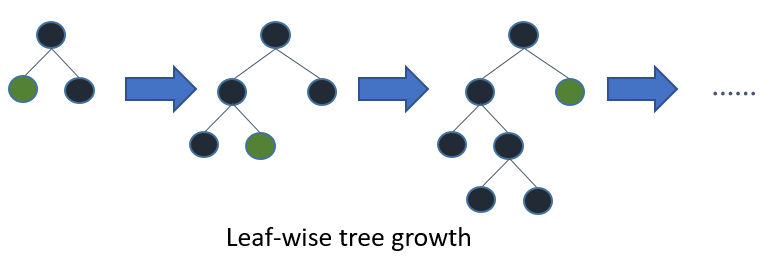# 每个模型如何处理分类变量？

## CatBoost

CatBoost 最大的优势是：对于离散特征特别多的数据，效果比较好，可以灵活地提供分类列（cat_features）的索引，以便可以使用one_hot_max_size将其编码为单热编码（对具有不同值数小于或等于给定参数值的所有要素使用独热编码）。
如果您没有cat_features参数中传递任何内容，CatBoost 会将所有列视为数值变量。此时效果可能不是很好。

注： 如果cat_features中未提供具有字符串值的列，CatBoost 将引发错误。此外，默认情况下，具有默认int类型的列将被视为数字，必须在cat_features中指定它以使算法将其视为分类。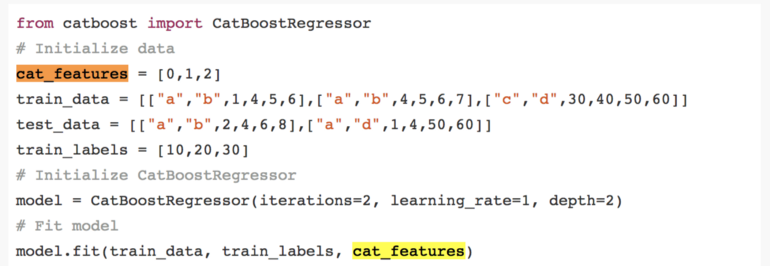这里cat_features=[0,1,2]表示输入的特征数据中，第1，2,3列数据是离散特征数据，而没有指定的4,5，6列数据就是数值型特征。
对于类别的唯一数量大于 one_hot_max_size 的其余分类列（也就是离散特征取值大于one_hot_max_size ，用onehot编码则维度增加特别高，比如‘’民族‘’特征有56个，onehot编码后，就有56维，计算量一下暴增。），CatBoost 使用一种有效的编码方法，该方法类似于平均编码，但可减少过度拟合。这个过程是这样的：

• 1、以随机顺序排列输入观测值集。也就是对数据进行重排序。生成多个随机排列

这里理解一下为什么要对数据进行随机重排序？因为catboost采用的编码方式和数据的先后顺序有关，所以把数据打乱后，会得到多份编码不同的数据，这个有点“数据增强”的意思。比如图片我们旋转后会得到不同的数据，catboost随机打乱数据，就有点异曲同工。
比如从一个高斯分布中采样得到3个点a=0.1，b=-0.2，c=0.3。如果不区分数据顺序，那么这就是一次采样，而如果采用catboost，随机排列，会出现[a,b,c],[b.a,c],[b,c,a],[a,c,b]等等3！=6份数据，相当于做了6次不同的采样，所以比起不考虑顺序的1次采样，catboost进行了数据增强，相当于同一个分布，采样了6次，这样catboost的精度以及过拟合都会有所提高。
这个技巧比较巧妙。

• 2、将标签值从浮点或类别转换为整数
• 3、使用以下公式将所有分类要素值转换为数值：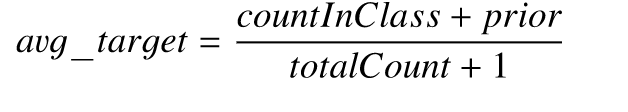其中，CountInClass 是具有和当前待编码的数据的特征值相同的数据，并且标签值等于“1”的次数， Prior 是分子的初步值，它由起始参数确定。TotalCount 是具有与当前特征值一样的数据的总数（截至当前对象。）

这里用一个示例解释一下，我们设 Prior =0.5.我们对第6行数据进行编码。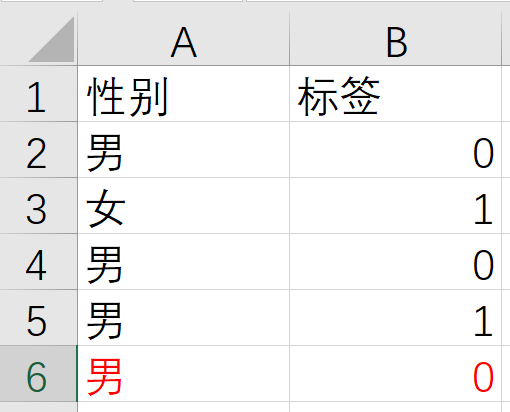TotalCount就是性别为男的数据，并且在待编码行（第六行）之前的数据，一共3条，分别是记录（2、4、5）。CountInClass 是之前特征为男性，并且标签为1的数据，就只有一行，就是第5行、所以TotalCount=3，CountInClass =1。
带入avg_target=（1+0.5）/(3+1)=0.375，因此，第六行的男这个值，会被替换为0.375。
在数学上，这可以用下面的等式来表示：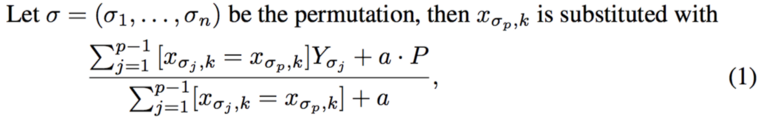## LightGBM

与CatBoost类似，LightGBM也可以通过输入特征名称来处理分类特征。它不会转换为独热编码，并且比独热编码快得多。LGBM使用特殊算法来查找分类特征的分割值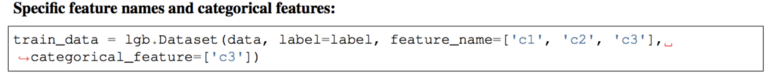注： 在为 LGBM 构造数据集之前，应将分类要素转换为 int 类型。它不接受字符串值，即使您通过categorical_feature参数传递字符串值也是如此。

## XGBoost

与CatBoost或LGBM不同，XGBoost本身无法处理分类特征，它只接受类似于随机森林的数值。因此，在向 XGBoost 提供分类数据之前，必须执行各种编码，如标签编码、平均编码或单热编码。

# 超参数中的相似性

所有这些模型都有很多参数需要调整，但我们只介绍重要的参数。以下是这些参数根据其功能和不同型号的对应参数的列表。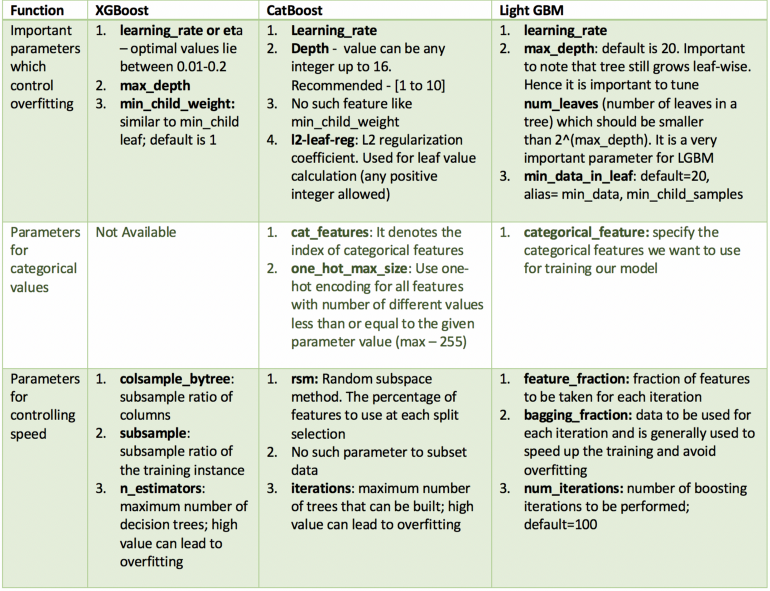# 数据集上的实现

我使用2015年航班延误的Kaggle数据集，因为它具有分类和数值特征。该数据集大约有 500 万行，将有利于判断每种类型增压的调优模型的速度和准确性的性能。我将采样使用此数据的10%子集〜500k行。不然训练太慢。
以下是用于建模的特征：

• 月、日、DAY_OF_WEEK：数据类型 int
• 航空公司和FLIGHT_NUMBER：数据类型 int
• ORIGIN_AIRPORT和DESTINATION_AIRPORT：数据类型字符串
• DEPARTURE_TIME：数据类型浮点型
• ARRIVAL_DELAY：这将是目标，并转换为布尔变量，指示延迟超过 10 分钟
• 距离和AIR_TIME：数据类型浮点型

## 数据预处理

import pandas as pd, numpy as np, time
from sklearn.model_selection import train_test_split, GridSearchCV
from sklearn import metrics

data = data.sample(frac=0.1, random_state=10)
print(data.columns)

data = data[["MONTH", "DAY", "DAY_OF_WEEK", "AIRLINE", "FLIGHT_NUMBER", "DESTINATION_AIRPORT",
"ORIGIN_AIRPORT", "AIR_TIME", "DEPARTURE_TIME", "DISTANCE", "ARRIVAL_DELAY"]]

data.dropna(inplace=True)

data["ARRIVAL_DELAY"] = (data["ARRIVAL_DELAY"] > 10) * 1

cols = ["AIRLINE", "FLIGHT_NUMBER", "DESTINATION_AIRPORT", "ORIGIN_AIRPORT"]
for item in cols:
data[item] = data[item].astype("category").cat.codes +1

train, test, y_train, y_test = train_test_split(data.drop(["ARRIVAL_DELAY"], axis=1), data["ARRIVAL_DELAY"],
random_state=10, test_size=0.25)


## XGBoost

import xgboost as xgb

def auc(m, train, test):
return (metrics.roc_auc_score(y_train,m.predict_proba(train)[:,1]),
metrics.roc_auc_score(y_test,m.predict_proba(test)[:,1]))

# Parameter Tuning
model = xgb.XGBClassifier(tree_method='gpu_hist', gpu_id=0)
param_dist = {"max_depth": [10,20,30],
"min_child_weight" : [1,3],
"n_estimators": ,
"learning_rate": [0.05, 0.1,0.5],}
grid_search = GridSearchCV(model, param_grid=param_dist, cv = 3,
verbose=10, n_jobs=-1)
grid_search.fit(train, y_train)

print(grid_search.best_estimator_)

model = xgb.XGBClassifier(max_depth=20, min_child_weight=3,  n_estimators=200,\
n_jobs=-1 , verbose=1,learning_rate=0.1
,tree_method='gpu_hist', gpu_id=0)
model.fit(train,y_train,verbose=10)

print('xgboost model score')
print(auc(model, train, test))
#xgboost model score (0.9999815408046491, 0.7848942647211888)


xgboost model score (0.9999815408046491, 0.7848942647211888)

## LGBM

import lightgbm as lgb
from sklearn import metrics

def auc2(m, train, test):
return (metrics.roc_auc_score(y_train, m.predict(train)),
metrics.roc_auc_score(y_test, m.predict(test)))

lg = lgb.LGBMClassifier(verbose=1
,device_type ='gpu')
param_dist = {"max_depth": [25,50, 75],
"learning_rate" : [0.01,0.05,0.1],
"num_leaves": [300,900,1200],
"num_iterations": 
}
grid_search = GridSearchCV(lg, n_jobs=-1, param_grid=param_dist, cv = 3, scoring="roc_auc", verbose=5)
grid_search.fit(train,y_train)
print('LGBMClassifier best_estimator:',grid_search.best_estimator_)

d_train = lgb.Dataset(train, label=y_train, free_raw_data=False)
# END learning_rate=0.05, max_depth=25, num_iterations=200, num_leaves=1200;, score=0.772 total time=10.6min
params = {"max_depth": 25, "learning_rate": 0.05, "num_leaves": 1200, "num_iterations": 200,
# 'device': "gpu",'gpu_device_id': 1,
#   'gpu_platform_id': 1,
#   'gpu_use_dp': 'false',
#   'max_bin': 252,
#   'num_gpu':1
}

# Without Categorical Features
model2 = lgb.train(params, d_train)
print('lightGBM Without Categorical Features:', auc2(model2, train, test))
# lightGBM Without Categorical Features: (0.9820454074519167, 0.7842988189129978)

# With Catgeorical Features
cate_features_name = ["MONTH", "DAY", "DAY_OF_WEEK", "AIRLINE", "DESTINATION_AIRPORT",
"ORIGIN_AIRPORT"]
model2 = lgb.train(params, d_train, categorical_feature=cate_features_name)
print('lightGBM With Categorical Features:', auc2(model2, train, test))
# lightGBM With Categorical Features: (0.9849578833000501, 0.7765037053711114)


lightGBM Without Categorical Features: (0.9820454074519167, 0.7842988189129978)

## catboost

在调整 CatBoost 的参数时，很难传递分类特征的索引。因此，我在不传递分类特征的情况下调整了参数，并评估了两个模型 :一个具有分类特征，另一个区分没有类别特征。我单独调整了one_hot_max_size，因为它不会影响其他参数。

import catboost

cat_features_index = [0, 1, 2, 3, 4, 5, 6]

def auc(m, train, test):
return (metrics.roc_auc_score(y_train, m.predict_proba(train)[:, 1]),
metrics.roc_auc_score(y_test, m.predict_proba(test)[:, 1]))

params = {'depth': [7, 10,13],
'learning_rate': [0.03, 0.15, 0.2],
'l2_leaf_reg': [1, 4, 7],
'iterations': [300,500]}
devices='0')
cb_model = GridSearchCV(cb, params, scoring="roc_auc", cv=3)
cb_model.fit(train, y_train)
print('CatBoostClassifier best_estimator:',cb_model.best_estimator_)
print('CatBoostClassifier best_params_:',cb_model.best_params_)

# With Categorical features
clf = catboost.CatBoostClassifier(eval_metric="AUC", depth=10, iterations=500, l2_leaf_reg=9, learning_rate=0.15
clf.fit(train, y_train,plot=True)
print('CatBoostClassifier Without Categorical Features:', auc(clf, train, test))
# CatBoostClassifier Without Categorical Features: (0.8411768106404672, 0.7571423657177548)

# With Categorical features
clf = catboost.CatBoostClassifier(eval_metric="AUC", one_hot_max_size=31, \
depth=10, iterations=300, l2_leaf_reg=4, learning_rate=0.15
clf.fit(train, y_train, cat_features=cat_features_index,plot=True)
print('CatBoostClassifier With Categorical Features:', auc(clf, train, test))
# CatBoostClassifier With Categorical Features: (0.881573635663068, 0.8129717796545255)


# 结果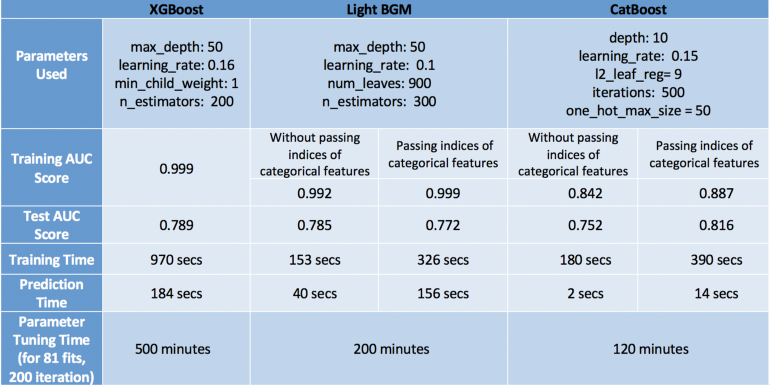# 总结

为了评估模型，我们应该从速度和精度的角度来研究模型的性能。

牢记这一点，CatBoost在测试集上以最大的精度（0.816），最小的过拟合（训练和测试精度都接近），以及最小的预测时间和调整时间成为赢家。但这仅仅是因为我们考虑了分类变量并调整了one_hot_max_size。如果我们不利用CatBoost的这些功能，它的表现最差，准确度仅为0.752。因此，我们了解到，只有当数据中有分类变量并正确调整它们时，CatBoost才会表现良好。

我们的下一个表演者是XGBoost，通常效果很好。它的准确性非常接近CatBoost，即使忽略了我们在数据中有分类变量的事实，我们已经将其转换为数值以供其使用。但是，XGBoost的唯一问题是它太慢了。特别是调整其参数真的很令人沮丧（我花了6个小时来运行GridSearchCV - 非常糟糕的主意！更好的方法是单独调整参数，而不是使用GridSearchCV。
提示：使用GPU可能会所有加快，默认算法使用cpu，因此本论文在原作者上改进使用GPU，会快不少。但目前lgbm不支持cuda，无法使用英伟达的显卡加速。

最后，最后一个地方是Light GBM。这里需要注意的一件重要事情是，当使用cat_features时，它在速度和准确性方面表现不佳。我相信它表现不佳的原因是因为它对分类数据使用了某种修改的均值编码，导致过度拟合（训练精度相当高 - 与测试精度相比为0.999）。然而，如果我们像XGBoost一样正常使用它，它可以达到与XGBoost（LGBM - 0.785，XGBoost- 0.789）更快的速度相似的精度。

最后，我不得不说，这些观察结果对于这个特定的数据集是正确的，对于其他数据集可能有效，也可能不有效。但是，有一件事是正确的，那就是：XGBoost比其他两种算法慢。

那么你最喜欢哪一个呢？请评论原因。
任何反馈或改进建议将不胜感激！

# 全部代码

import matplotlib.pyplot as plt
import pandas as pd, numpy as np, time
from sklearn.model_selection import train_test_split, GridSearchCV
from sklearn import metrics

print('data.shape:',data.shape)
data = data.sample(frac=0.1, random_state=10)
print('after sample data.shape:',data.shape)
print(data.columns)

data = data[["MONTH", "DAY", "DAY_OF_WEEK", "AIRLINE", "FLIGHT_NUMBER", "DESTINATION_AIRPORT",
"ORIGIN_AIRPORT", "AIR_TIME", "DEPARTURE_TIME", "DISTANCE", "ARRIVAL_DELAY"]]

data.dropna(inplace=True)

data["ARRIVAL_DELAY"] = (data["ARRIVAL_DELAY"] > 10) * 1

cols = ["AIRLINE", "FLIGHT_NUMBER", "DESTINATION_AIRPORT", "ORIGIN_AIRPORT"]
for item in cols:
data[item] = data[item].astype("category").cat.codes + 1

train, test, y_train, y_test = train_test_split(data.drop(["ARRIVAL_DELAY"], axis=1), data["ARRIVAL_DELAY"],
random_state=10, test_size=0.25)

import xgboost as xgb

def auc(m, train, test):
return (metrics.roc_auc_score(y_train, m.predict_proba(train)[:, 1]),
metrics.roc_auc_score(y_test, m.predict_proba(test)[:, 1]))

# # Parameter Tuning
# model = xgb.XGBClassifier(tree_method='gpu_hist', gpu_id=0)
# param_dist = {"max_depth": [10,20,30],
#               "min_child_weight" : [1,3],
#               # "n_estimators": [200,500],
#               "subsample":[0.8,0.9,1],
#               "lambda":[3,6,9],
#               "alpha":[3,6],
#               # "learning_rate": [0.05, 0.1,0.5],
#                "learning_rate": [0.1],
#               }
# grid_search = GridSearchCV(model, param_grid=param_dist, cv = 3,
#                                    verbose=10, n_jobs=-1)
# grid_search.fit(train, y_train)
# print('XGBClassifier best_params_:', grid_search.best_params_)
# # alpha=3, lambda=3, learning_rate=0.1, max_depth=20, min_child_weight=1,
# # subsample=0.8;, score=0.810 total time=38.6min

model = xgb.XGBClassifier(max_depth=20, min_child_weight=3,  n_estimators=1000,learning_rate=0.05,
reg_lambda=1, alpha=1,
# subsample=0.8,
n_jobs=-1, verbose=10,
tree_method='gpu_hist', gpu_id=0)
model.fit(train,y_train,verbose=10)

print('xgboost model score:',auc(model, train, test))
#xgboost model score (0.9999999975811984, 0.7899954118937722)
#20% xgboost model score: (0.9999985093055754, 0.8220708882482494)

import lightgbm as lgb
def auc2(m, train, test):
return (metrics.roc_auc_score(y_train, m.predict(train)),
metrics.roc_auc_score(y_test, m.predict(test)))

# lg = lgb.LGBMClassifier(verbose=0,
#                         learning_rate=0.5,
#                         device_type='gpu',gpu_device_id= 0,gpu_platform_id=0,gpu_use_dp='false')
# param_dist = {
#     "max_depth": [25, 50, 75],
#     #           "learning_rate": [0.01, 0.05, 0.1],
#               "num_leaves": [100,300, 900],
#     #           "num_iterations": [200,500],
#     #           "feature_fraction": [0.7,0.8, 0.9, 1.0],
#     #           "bagging_fraction": [0,7,0.8, 0.9, 1.0],
#     # LGBMClassifier best_params_: {'bagging_fraction': 0.8, 'feature_fraction': 1.0}
#             'bagging_fraction': [0.8], 'feature_fraction': [1.0],
#
#               # "lambda_l1": [0, 3, 6, 9],
#               # "lambda_l2": [0, 3, 6],
#     # LGBMClassifier best_params_: {'lambda_l1': 6, 'lambda_l2': 3, 'num_iterations': 500}
#             'lambda_l1': , 'lambda_l2': , 'num_iterations': ,
#     # LGBMClassifier best_params_: {'bagging_fraction': 0.8, 'feature_fraction': 1.0,
#     # 'lambda_l1': 6, 'lambda_l2': 3, 'max_depth': 75, 'num_iterations': 500, 'num_leaves': 300}
#               }
# grid_search = GridSearchCV(lg, n_jobs=-1, param_grid=param_dist, cv=3, scoring="roc_auc", verbose=1)
# grid_search.fit(train, y_train)
# print('LGBMClassifier best_estimator:', grid_search.best_estimator_)
# print('LGBMClassifier best_params_:', grid_search.best_params_)
# # learning_rate=0.05, max_depth=50, num_iterations=200, num_leaves=300;, score=0.763 total time= 3.1min

d_train = lgb.Dataset(train, label=y_train, free_raw_data=False)
# END learning_rate=0.05, max_depth=25, num_iterations=200, num_leaves=1200;, score=0.772 total time=10.6min
params = {"max_depth": 75, "learning_rate": 0.1, "num_leaves": 300,
'lambda_l1': 6, 'lambda_l2': 3, 'num_iterations': 2000,
'bagging_fraction': 0.8, 'feature_fraction': 1.0,
# 'device': "gpu", 'gpu_device_id': 1,
# 'gpu_platform_id': 1,
# 'gpu_use_dp': 'false',
# 'max_bin': 252,
# 'num_gpu': 2
}

# Without Categorical Features
model2 = lgb.train(params, d_train)
print('lightGBM Without Categorical Features:', auc2(model2, train, test))
# lightGBM Without Categorical Features: (0.9832532745589256, 0.7892403986379332)
#
# With Catgeorical Features
cate_features_name = ["MONTH", "DAY", "DAY_OF_WEEK", "AIRLINE", "DESTINATION_AIRPORT",
"ORIGIN_AIRPORT"]
model2 = lgb.train(params, d_train, categorical_feature=cate_features_name)
print('lightGBM With Categorical Features:', auc2(model2, train, test))
# lightGBM With Categorical Features: (0.9849578833000501, 0.7765037053711114)

import catboost

cat_features_index = [0, 1, 2, 3, 4, 5, 6]

def auc(m, train, test):
return (metrics.roc_auc_score(y_train, m.predict_proba(train)[:, 1]),
metrics.roc_auc_score(y_test, m.predict_proba(test)[:, 1]))

#
# # params = {'depth': [7, 10,13],
# #           'learning_rate': [0.03, 0.15, 0.2],
# #           'l2_leaf_reg': [1, 4, 7],
# #           'iterations': [300,500]}
# # cb = catboost.CatBoostClassifier(task_type="GPU",
# #                            devices='0')
# # cb_model = GridSearchCV(cb, params, scoring="roc_auc", cv=3)
# # cb_model.fit(train, y_train)
# # print('CatBoostClassifier best_estimator:',cb_model.best_estimator_)
# # print('CatBoostClassifier best_params_:',cb_model.best_params_)
#
# With Categorical features
clf = catboost.CatBoostClassifier(eval_metric="AUC", depth=10, iterations=500, l2_leaf_reg=4, learning_rate=0.15
clf.fit(train, y_train,plot=True)
print('CatBoostClassifier Without Categorical Features:', auc(clf, train, test))
# CatBoostClassifier Without Categorical Features: (0.8411768106404672, 0.7571423657177548)
# 20% CatBoostClassifier Without Categorical Features: (0.8125473336833896, 0.767777462764133)

# With Categorical features
clf = catboost.CatBoostClassifier(eval_metric="AUC", one_hot_max_size=31, \
depth=10, iterations=500, l2_leaf_reg=4, learning_rate=0.15
clf.fit(train, y_train, cat_features=cat_features_index,plot=True)
print('CatBoostClassifier With Categorical Features:', auc(clf, train, test))
plt.show()
# CatBoostClassifier With Categorical Features: (0.881573635663068, 0.8129717796545255)
# CatBoostClassifier With Categorical Features: (0.8969051893380249, 0.8243120308981184)
# 20% CatBoostClassifier With Categorical Features: (0.8942350930660874, 0.8478804417411229)


展开全文机器学习 python
• ## catboost原理

千次阅读 多人点赞 2020-02-20 21:32:24
文章目录概述原理类别型特征类别型特征的相关工作目标变量统计（Target Statistics）CatBoost处理Categorical features总结梯度偏差/预测偏移为什么会有梯度偏差？梯度偏差造成了什么问题？如何解决梯度偏差/预测...

# 概述

CatBoost是俄罗斯的搜索巨头Yandex在2017年开源的机器学习库，是Boosting族算法的一种。CatBoost和XGBoost、LightGBM并称为GBDT的三大主流神器，都是在GBDT算法框架下的一种改进实现。XGBoost被广泛的应用于工业界，LightGBM有效的提升了GBDT的计算效率，而Yandex的CatBoost号称是比XGBoost和LightGBM在算法准确率等方面表现更为优秀的算法。

CatBoost是一种基于对称决策树（oblivious trees）为基学习器实现的参数较少、支持类别型变量和高准确性的GBDT框架，主要解决的痛点是高效合理地处理类别型特征，CatBoost是由Categorical和Boosting组成。此外，CatBoost还解决了梯度偏差（Gradient Bias）及预测偏移（Prediction shift）的问题，从而减少过拟合的发生，进而提高算法的准确性和泛化能力。

与XGBoost、LightGBM相比，CatBoost的创新点有：

• 嵌入了自动将类别型特征处理为数值型特征的创新算法。首先对categorical features做一些统计，计算某个类别特征（category）出现的频率，之后加上超参数，生成新的数值型特征（numerical features）。
• Catboost还使用了组合类别特征，可以利用到特征之间的联系，这极大的丰富了特征维度。
• 采用ordered boost的方法避免梯度估计的偏差，进而解决预测偏移的问题。
• 采用了完全对称树作为基模型。

# 原理

## 类别型特征的相关工作

所谓类别型特征，即这类特征不是数值型特征，而是离散的集合，比如省份名（山东、山西、河北等），城市名（北京、上海、深圳等），学历（本科、硕士、博士等）。在梯度提升算法中，最常用的是将这些类别型特征转为数值型来处理，一般类别型特征会转化为一个或多个数值型特征。

• 如果某个类别型特征基数比较低（low-cardinality features），即该特征的所有值去重后构成的集合元素个数比较少，一般利用One-hot编码方法将特征转为数值型。One-hot编码可以在数据预处理时完成，也可以在模型训练的时候完成，从训练时间的角度，后一种方法的实现更为高效，CatBoost对于基数较低的类别型特征也是采用后一种实现。

• 高基数类别型特征（high cardinality features） 当中，比如 user ID，这种编码方式会产生大量新的特征，造成维度灾难。一种折中的办法是可以将类别分组成有限个的群体再进行One-hot编码。上述方法的一种常用解决方法是根据目标变量统计（Target Statistics，以下简称TS）进行分组，目标变量统计用于估算每个类别的目标变量期望值。甚至有人直接用TS作为一个新的数值型变量来代替原来的类别型变量

• 增加计算时间，因为需要对每一个类别型特征，在迭代的每一步，都需要对GS进行计算；
• 增加存储需求，对于一个类别型变量，需要存储每一次分离每个节点的类别；

为了克服这些缺点，LightGBM以损失部分信息为代价将所有的长尾类别归为一类，作者声称这样处理高基数类别型特征时比One-hot编码还是好不少。另外对于采用TS特征，仅仅为每个类别计算和存储一个数字。

采用TS作为一个新的数值型特征是最有效、信息损失最小的处理类别型特征的方法。TS也被广泛应用在点击预测任务当中，这个场景当中的类别型特征有用户、地区、广告、广告发布者等。

### 目标变量统计（Target Statistics）

1. CatBoost算法的设计初衷是为了更好的处理GBDT特征中的categorical features。在处理 GBDT特征中的categorical features的时候，最简单的方法是用 categorical feature 对应的标签的平均值来替换。在决策树中，标签平均值将作为节点分裂的标准。这种方法被称为 Greedy Target-based Statistics , 简称 Greedy TS，用公式来表达就是：
x i , k = ∑ j = 1 n [ x j , k = x i , k ] ⋅ Y j ∑ j = 1 n [ x j , k = x i , k ] x_{i,k} = \frac{\sum\limits_{j=1}^n[x_{j,k}=x_{i,k}]\cdot Y_j}{\sum\limits_{j=1}^n[x_{j,k}=x_{i,k}]}
这种方法有一个显而易见的缺陷，就是通常特征比标签包含更多的信息，如果强行用标签的平均值来表示特征的话，当训练数据集和测试数据集数据结构和分布不一样的时候会出条件偏移问题。

2. 一个标准的改进 Greedy TS的方式是添加先验分布项，这样可以减少噪声和低频率类别型数据对于数据分布的影响
x i , k = ∑ j = 1 p − 1 [ x σ j , k = x σ p , k ] ⋅ Y j + a ⋅ p ∑ j = 1 p − 1 [ x σ j , k = x σ p , k ] + a x_{i,k} = \frac{\sum\limits_{j=1}^{p-1}[x_{\sigma_{j,k}}=x_{\sigma_{p,k}}]\cdot Y_j + a\cdot p}{\sum\limits_{j=1}^{p-1}[x_{\sigma_{j,k}}=x_{\sigma_{p,k}}] + a}

其中p是添加的先验项，a通常是大于0的权重系数。添加先验项是一个普遍做法，针对类别数较少的特征，它可以减少噪声数据。。对于回归问题，一般情况下，先验项可取数据集label的均值。对于二分类，先验项是正例的先验概率。
当然，在论文《CatBoost: unbiased boosting with categorical features》中，还提到了其它几种改进Greedy TS的方法，分别有：Holdout TS、Leave-one-out TS、Ordered TS。我这里就不再翻译论文中的这些方法了，感兴趣的同学可以自己翻看一下原论文。

1. Ordered TS
它是catboost的主要思想，依赖于排序，受online learning algorithms的启发得到，对于某一个样本，TS的值依赖于观测历史，为了在离线的数据上应用该思想，我们将数据随机排序，对于每一个样本，利用该样本之前数据计算该样本类别值的TS值。如果仅仅使用一个随机序列，那么计算得到值会有较大的方差，因此我们使用不同的随机序列来计算。

### CatBoost处理Categorical features总结

• 首先会计算一些数据的statistics。计算某个category出现的频率，加上超参数，生成新的numerical features。这一策略要求同一标签数据不能排列在一起（即先全是之后全是这种方式），训练之前需要打乱数据集。
• 第二，使用数据的不同排列。在每一轮建立树之前，先扔一轮骰子，决定使用哪个排列来生成树。
• 第三，考虑使用categorical features的不同组合。例如颜色和种类组合起来，可以构成类似于blue dog这样的特征。当需要组合的categorical features变多时，CatBoost只考虑一部分combinations。在选择第一个节点时，只考虑选择一个特征，例如A。在生成第二个节点时，考虑A和任意一个categorical feature的组合，选择其中最好的。就这样使用贪心算法生成combinations。
• 第四，除非向gender这种维数很小的情况，不建议自己生成One-hot编码向量，最好交给算法来处理。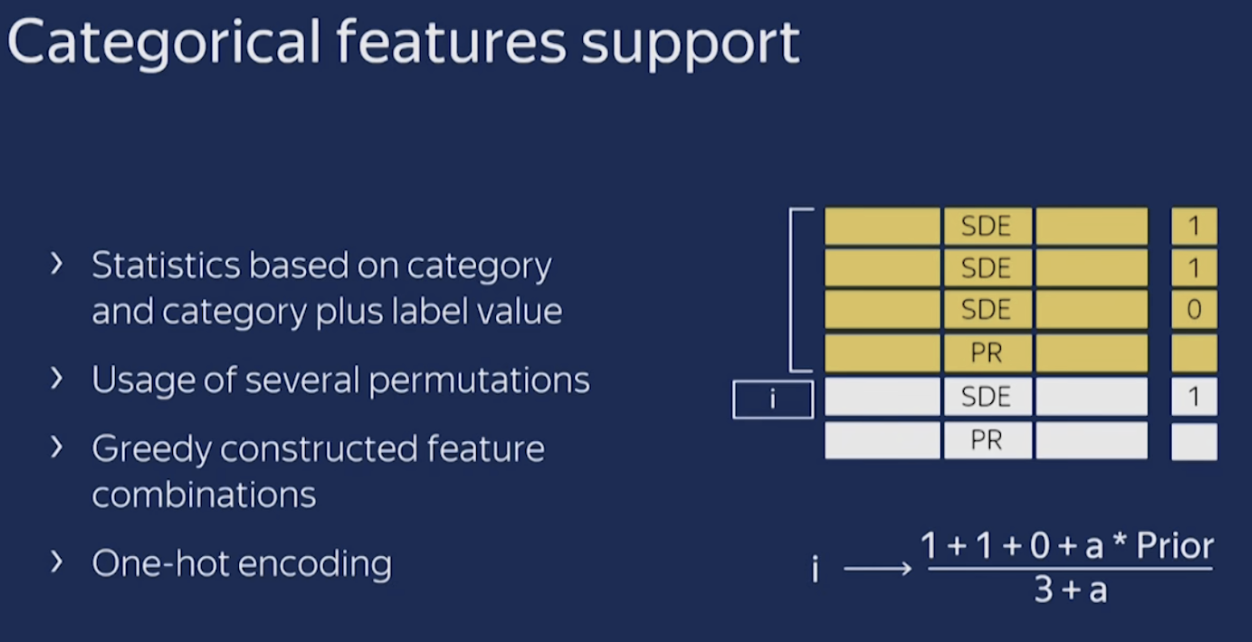## 梯度偏差/预测偏移

1. 为什么会有梯度偏差？
2. 梯度偏差造成了什么问题？
3. 如何解决梯度偏差？

### 为什么会有梯度偏差？

CatBoost和所有标准梯度提升算法一样，都是通过构建新树来拟合当前模型的梯度。然而，所有经典的提升算法都存在由有偏的点态梯度估计引起的过拟合问题。在每个步骤中使用的梯度都使用当前模型中的相同的数据点来估计，这导致估计梯度在特征空间的任何域中的分布与该域中梯度的真实分布相比发生了偏移。

### 梯度偏差造成了什么问题？

模型过拟合，预测发生偏移。另外预测偏移还有当我们利用TS来处理类别特征时，引起的target leak的问题。

### 如何解决梯度偏差/预测偏移？

为了解决这个问题，CatBoost对经典的梯度提升算法进行了一些改进，简要介绍如下:

许多利用GBDT技术的算法（例如，XGBoost、LightGBM），构建下一棵树分为两个阶段：选择树结构和在树结构固定后计算叶子节点的值。为了选择最佳的树结构，算法通过枚举不同的分割，用这些分割构建树，对得到的叶子节点计算值，然后对得到的树计算评分，最后选择最佳的分割。两个阶段叶子节点的值都是被当做梯度或牛顿步长的近似值来计算。

在CatBoost中，第一阶段采用梯度步长的无偏估计，第二阶段使用传统的GBDT方案执行。既然原来的梯度估计是有偏的，那么怎么能改成无偏估计呢？

F i F^i 为构建i棵树后的模型, g i ( X k , y k ) g^i(X_k, y_k) 为构建i棵树后第k个训练样本上面的梯度值。为了使得 g i ( X k , y k ) g^i(X_k, y_k) 无偏于模型 F i F^i ，我们需要在没有 X k X_k 参与的情况下对模型 F i F^i 进行训练。我们需要对所有训练样本计算无偏的梯度估计

我们可以运用下面这个技巧来处理这个问题：对于每一个样本 X k X_k ，我们训练一个单独的模型 M k M_k (该模型由多颗树组成)，且该模型从不使用基于该样本的梯度估计进行更新。我们使用 M k M_k 估计上 X k X_k 的梯度（即叶子节点的值），并使用这个估计对结果树进行评分。用伪码描述如下，其中 L o s s ( y i , a ) Loss(y_i, a) 是需要优化的损失函数，y是标签值，a是公式计算值。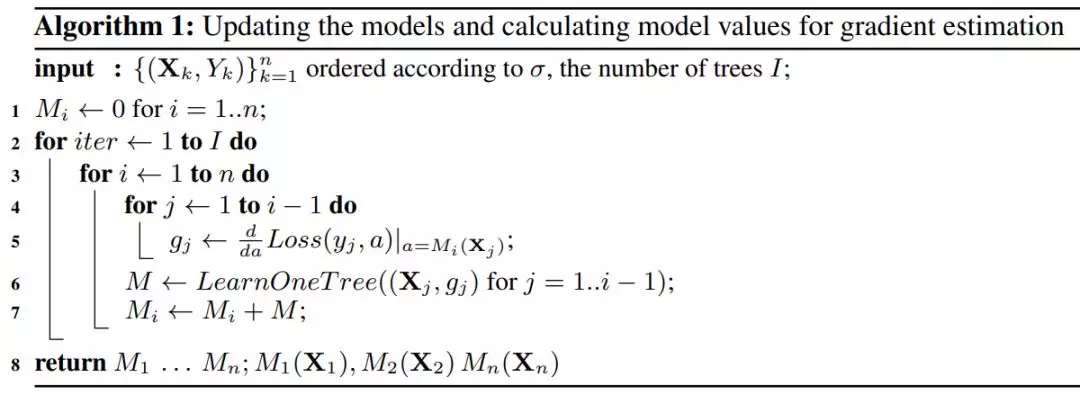值得注意的是模型的建立并没有 X i X_i 样本的参与，并且CatBoost中所有的树的共享同样的结构。 M i ( X i ) M_i(X_i) 表示模型 M i M_i X i X_i 进行打分。

这里为了进一步减少过拟合，会使用若干不同对扰动来加强算法鲁棒性。也即上述提到对用于计算类别特征的方法，我们获取s个随机扰动排列，对于每个排列，我们又训练n个不同的模型 M i M_i ，这样模型模型复杂度为 O ( s n 2 ) O(sn^2) ，后续进行优化，具体见算法流程部分；

## 其他

### 特征组合

CatBoost的另外一项重要实现是将不同类别型特征的组合作为新的特征，以获得高阶依赖（high-order dependencies），比如在广告点击预测当中用户ID与广告话题之间的联合信息，又或者在音乐推荐引用当中，用户ID和音乐流派，如果有些用户更喜欢摇滚乐，那么将用户ID和音乐流派分别转换为数字特征时，这种用户内在的喜好信息就会丢失。然而，组合的数量会随着数据集中类别型特征的数量成指数增长，因此在算法中考虑所有组合是不现实的。为当前树构造新的分割点时，CatBoost会采用贪婪的策略考虑组合。对于树的第一次分割，不考虑任何组合。对于下一个分割，CatBoost将当前树的所有组合、类别型特征与数据集中的所有类别型特征相结合，并将新的组合类别型特征动态地转换为数值型特征。CatBoost还通过以下方式生成数值型特征和类别型特征的组合：树中选定的所有分割点都被视为具有两个值的类别型特征，并像类别型特征一样地被进行组合考虑。

当特征组合由原始的1到2时，logloss的logloss部分提升(logloss实际数值降低的)为1.86%，从1到3提升2.04%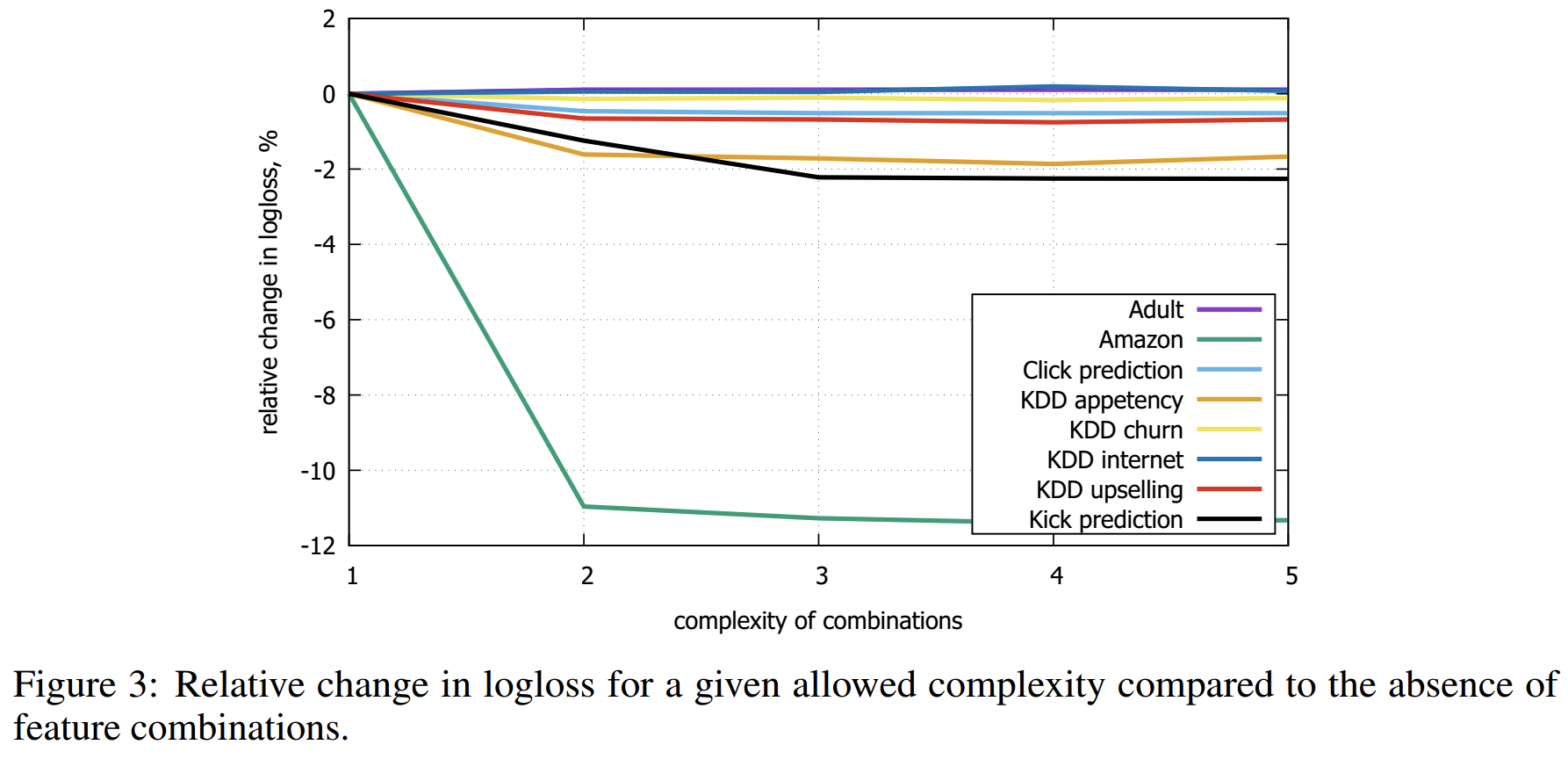### 快速评分

CatBoost使用对称树（oblivious trees）作为基预测器。在这类树中，相同的分割准则在树的整个一层上使用。这种树是平衡的，不太容易过拟合。梯度提升对称树被成功地用于各种学习任务中。在对称树中，每个叶子节点的索引可以被编码为长度等于树深度的二进制向量。这在CatBoost模型评估器中得到了广泛的应用：我们首先将所有浮点特征、统计信息和独热编码特征进行二值化，然后使用二进制特征来计算模型预测值。

### 基于GPU实现快速训练

密集的数值特征。 对于任何GBDT算法而言，最大的难点之一就是搜索最佳分割。尤其是对于密集的数值特征数据集来说，该步骤是建立决策树时的主要计算负担。CatBoost使用oblivious 决策树作为基模型，并将特征离散化到固定数量的箱子中以减少内存使用。就GPU内存使用而言，CatBoost至少与LightGBM一样有效。主要改进之处就是利用了一种不依赖于原子操作的直方图计算方法。
类别型特征。 CatBoost实现了多种处理类别型特征的方法，并使用完美哈希来存储类别型特征的值，以减少内存使用。由于GPU内存的限制，在CPU RAM中存储按位压缩的完美哈希，以及要求的数据流、重叠计算和内存等操作。通过哈希来分组观察。在每个组中，我们需要计算一些统计量的前缀和。该统计量的计算使用分段扫描GPU图元实现。
多GPU支持。 CatBoost中的GPU实现可支持多个GPU。分布式树学习可以通过数据或特征进行并行化。CatBoost采用多个学习数据集排列的计算方案，在训练期间计算类别型特征的统计数据。

## 算法流程

建树的流程：

开始阶段：CatBoost对训练集随机生成s+1个不同对序列，其中 σ 1 , σ 2 , . . . , σ n \sigma_1, \sigma_2,...,\sigma_n 用于定义树结构，分裂节点的计算。 σ 0 \sigma_0 用于对生成树结构选择叶子节点对值。
对于测试集合，我们根据整个训练集的TS计算相应类别的值

在catboost中，基分类器是对称树，该类树平衡，能够减弱过拟合，加速预测，整体流程为：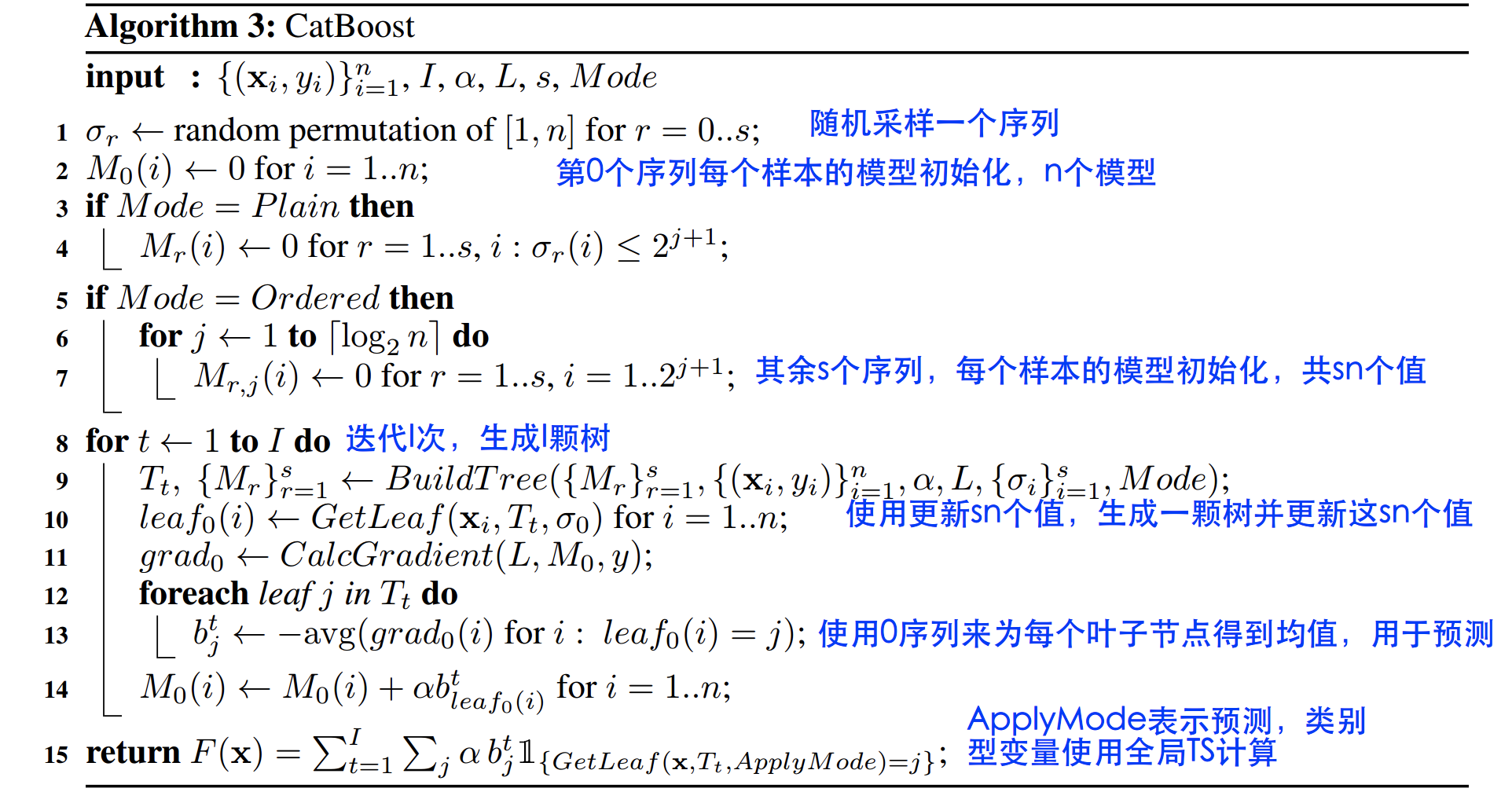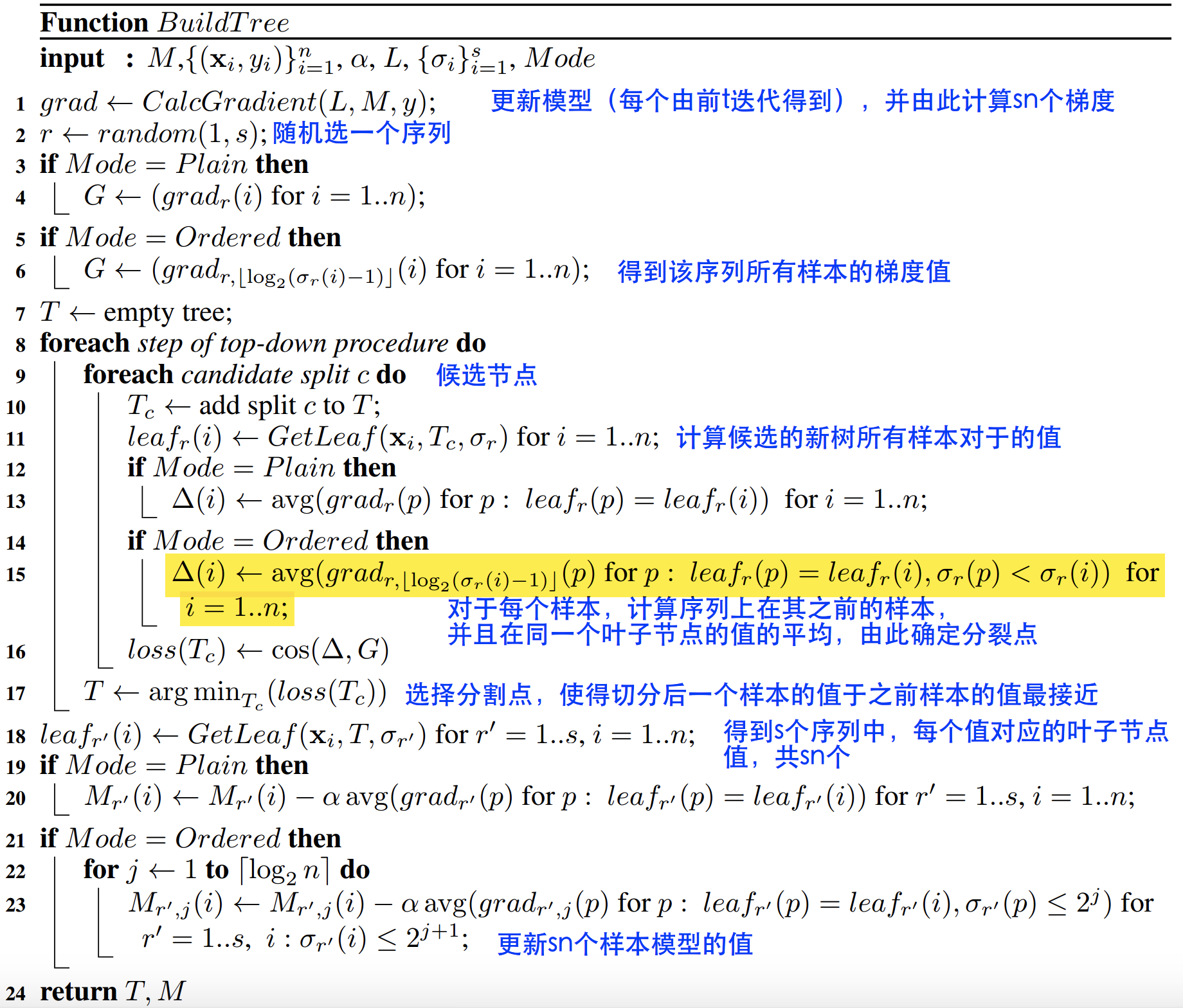• ordered boosting
ordered boosting解决类类别处理及梯度偏差引起的预测偏移问题， 开始提到的方法会训练n个模型，这也会n倍地增加了时间复杂度，为了简化，我们优化其时间复杂度，仅仅储存和计算 M r , j ′ ( i ) : = M , 2 j 其 中 j = 1 , . . . , [ l o g 2 n ] M'_{r,j}(i):=M_{,2^j} 其中 j=1,...,[log_2 n] ， 满足 σ r ( i ) ≤ 2 j + 1 \sigma_r(i) \leq 2^{j+1} 的i，这样总体时间复杂度由 O ( s n 2 ) O(sn^2) 转化为 O ( s n ) O(sn)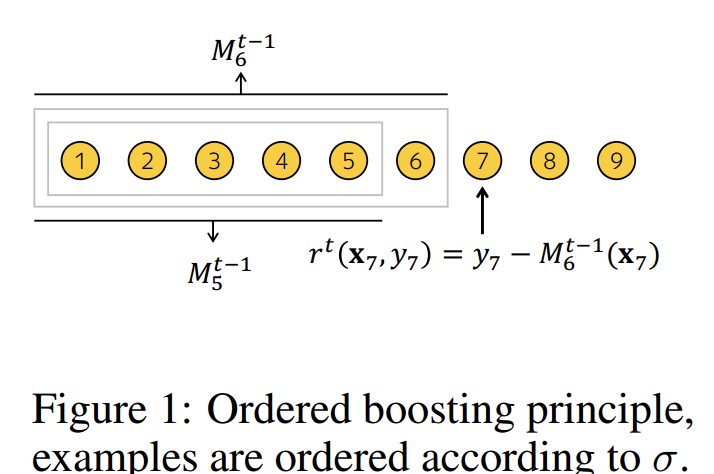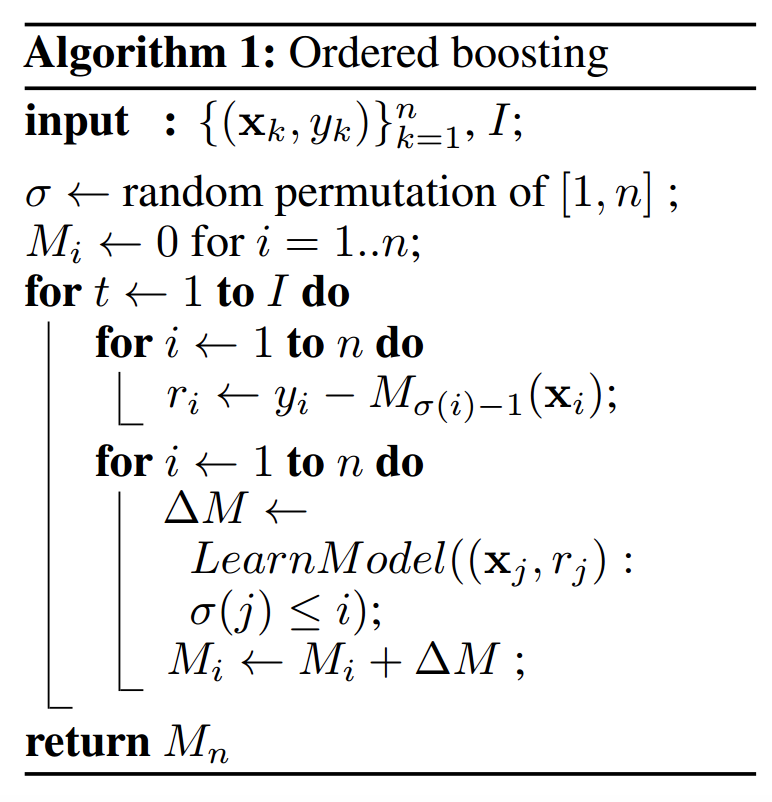# 总结

## 优点

• 性能卓越： 在性能方面可以匹敌任何先进的机器学习算法；
• 鲁棒性/强健性： 无需调参即可获得较高的模型质量，采用默认参数就可以获得非常好的结果，减少在调参上面花的时间,减少了对很多超参数调优的需求
• 易于使用： 提供与scikit集成的Python接口，以及R和命令行界面；
• 实用： 可以处理类别型、数值型特征，支持类别型变量，无需对非数值型特征进行预处理
• 可扩展： 支持自定义损失函数；
• 快速、可扩展的GPU版本，可以用基于GPU的梯度提升算法实现来训练你的模型，支持多卡并行提高准确性，
• 快速预测：即便应对延时非常苛刻的任务也能够快速高效部署模型

## 缺点

• 对于类别型特征的处理需要大量的内存和时间；
• 不同随机数的设定对于模型预测结果有一定的影响；

Ref:

1. Prokhorenkova, L., Gusev, G., Vorobev, A., Dorogush, A. V. & Gulin, A. CatBoost: unbiased boosting with categorical features. arXiv:1706.09516 [cs] (2017).
2. Dorogush, A. V., Ershov, V. & Gulin, A. CatBoost: gradient boosting with categorical features support.
3. CatBoost算法梳理
4. 深入理解catboost
展开全文• （3）支持Python或R的API接口来使用CatBoost，包括公式分析和训练可视化工具。 （4）有很多机器学习库的代码质量比较差，需要做大量的调优工作，而CatBoost只需少量调试，就可以实现良好的性能。这是一个关键性的机器学习 数据挖掘 python
• 数据特征工程 | Python实现CatBoost特征处理及选择机器学习 CatBoost
• ## 详解 CatBoost 原理

千次阅读 2022-04-07 15:47:34
详解 CatBoost 原理 集成学习的两大准则：基学习器的准确性和多样性。 算法：串行的Boosting和并行的Bagging,前者通过错判训练样本重新赋权来重复训练，来提高基学习器的准确性，降低偏差！后者通过采样方法，训练出...python 机器学习
• sklearn实战-乳腺癌细胞数据挖掘（博主亲自录制视频） ...utm_campaign=commission&utm_source=cp-400000000398149&utm_medium=share CatBoost En...
• 集成学习的两大准则：基学习器的准确性和多样性。 算法：串行的Boosting和...参数详解2.1通用参数：2.2默认参数2.3性能参数2.4参数调优3.CatBoost实战应用3.1回归案例3.2使用Pool加载数据集并进行预测3.3多分类案例..python 机器学习 人工智能
• 背景: 解决问题：对类别特征使用catboost，观察其特征重要程度。 今天搜索catboost的实例应用的博客，该博客的样例、demo、以及特征重要度打印，这三个内容，都是我想使用的。可是，他收费了，碰见我这个身无分文的...大数据
• catboost原论文，方便自己使用，也同时方便大家的使用，其实网络上也很好找的，这个可能不是随时都方便的。如有侵权，联系删除。
• ## catboost介绍

千次阅读 2021-09-05 15:50:30
一、Catboost简介 全称：Gradient Boosting(梯度提升) + Categorical Features(类别型特征) 作者：俄罗斯的搜索巨头Yandex 官方地址 论文链接 | 项目地址  文档地址 视频 二、Catboost的特点 　一般来说，...神经网络 机器学习
• Catboost已与交叉验证一起使用。 scikit-learn的train_test_split用于进行70％的训练，15％的验证和15％的测试数据。 对于每个kfold交叉验证，都使用catboost训练模型。 f1_score用于检查每个折叠的模型准确性。...
• 近几十年来，人们生活水平显著提高，...本文通过分析糖尿病的特点，针对医疗数据样本量小、容易缺失的特点，选择IV值分析进行特征选择、使用一种新型的Boosting算法CatBoost进行糖尿病患者预测，取得了显著的预测效果.
• ## quick test with AUC X_tr, X_val, y_tr, y_val = train_test_split(X_less_nas, y, test_size=0.2, random_state=SEED,stratify = y) cat_params = { 'loss_function': 'Logloss',...
• 最近，接触到一个比较新颖的Boost方法的机器... CatBoost是俄罗斯的搜索巨头Yandex在2017年开源的机器学习库，详细的介绍网上很多，这里就不多累赘了，Yandex的搜索入口如下：  https://tech.yandex.com/  官方...
• 对于类别型变量而言，xgb需要先自行...catboost进一步处理，不仅嵌入了对类别型变量的处理，并附带类别型特征交叉功能、还加入了部分文本数据的处理。本文深入浅出地详解catboost，全篇通俗易懂帮助大家掌握原理。...python 人工智能
• 使用TensorFlow和CatBoost的野火和野火热点检测系统。 该程序首先使用图像分类（Keras CNN）来识别给定卫星图像中是否存在野火。 如果未检测到火灾，则应用程序将向国家海洋和大气管理局查询周围区域和给定时间的...Python
• catboost算法预测房价在本项目中，我们使用不同类型的算法。 1）XGBOOST 2）LGBOOST 3）CatBoost 4）随机森林脚步： 1）从kaggle导入数据集2）预处理数据3）标签编码4）训练/测试分裂5）特征提取5.1）轻度渐变...
• CatBoost是一种基于对称决策树（oblivious trees）为基学习器实现的参数较少、支持类别型变量和高准确性的GBDT框架，主要解决的痛点是高效合理地处理类别型特征，这一点从它的名字中可以看出来，CatBoost是由...catboost catboost参数 catboost调参 catboost使用
• ## 30分钟学会CatBoost

千次阅读 2022-01-24 01:17:22
一，CatBoost简介 CatBoost和XGBoost、LightGBM并称为GBDT的三大主流神器，都是在GBDT算法框架下的一种改进实现。正如其名字所说那样，CatBoost主要是...python 机器学习 人工智能
• 本教程介绍了使用CatBoost的一些基本情况，例如模型训练，交叉验证和预测，以及一些有用的功能，如提早停止，快照支持，功能重要性和参数调整。 本教程中有17个问题。 尝试回答所有这些问题，这将帮助您学习如何...
• 我们知道，CatBoost可以很好地处理类别型数据。然而，它还具有大量的训练参数，可以更好地对类别型特征进行预处理。本文中，小猴子将和大家一起学习如何使用这些参数处理类别型特征的。CatBoost是一个开放源码的梯度...大数据 python 机器学习 人工智能
• sklearn实战-乳腺癌细胞数据挖掘（博主亲自录制视频） ...utm_campaign=commission&utm_source=cp-400000000398149&utm_medium=share catboost官网文档...
• CatBoost是一个快速，高性能的开放源代码库，可用于增强决策树的梯度。 这是一种具有大量应用程序的机器学习方法，包括针对Python，R，Java，C ++的排名，分类，回归和其他机器学习任务。 与许多数据集上的其他GBDT...
• 1 CatBoost简介 参考论文 Anna Veronika Dorogush, Andrey Gulin, Gleb Gusev, Nikita Kazeev, Liudmila Ostroumova Prokhorenkova, Aleksandr Vorobev "Fighting biases with dynamic boosting". arXiv:1706.09516...
• 在本文中，我们将仔细研究一个名为CatBoost的梯度增强库。在梯度提升中，预测是由一群弱学习者做出的。与为每个样本创建决策树的随机森林不同，在梯度增强中，树是一个接一个地创建的。模型中...决策树 机器学习 人工智能 python
• According to Catboost's official tutorial, it's good transform our NaN values to some number way out their distribution https://github.com/catboost/tutorials/blob/master/python_tutorial.ipynb ......